# Engineering salaries revisited

For a couple of posts, I have analysed what predictors affect the education level in Sweden. In this post, I will return to analysing the salary of engineers and I will try to use my experiences from studying the education level.

Statistics Sweden use NUTS (Nomenclature des Unités Territoriales Statistiques), which is the EU’s hierarchical regional division, to specify the regions.

Please send suggestions for improvement of the analysis to .

First, define libraries and functions.

library (tidyverse)
## -- Attaching packages -------------------------------------------------- tidyverse 1.3.0 --
## v ggplot2 3.2.1     v purrr   0.3.3
## v tibble  2.1.3     v dplyr   0.8.3
## v tidyr   1.0.2     v stringr 1.4.0
## v readr   1.3.1     v forcats 0.4.0
## -- Conflicts ----------------------------------------------------- tidyverse_conflicts() --
## x dplyr::lag()    masks stats::lag()
library (broom)
library (car)
## Loading required package: carData
##
## Attaching package: 'car'
## The following object is masked from 'package:dplyr':
##
##     recode
## The following object is masked from 'package:purrr':
##
##     some
library (sjPlot)
## Registered S3 methods overwritten by 'lme4':
##   method                          from
##   cooks.distance.influence.merMod car
##   influence.merMod                car
##   dfbeta.influence.merMod         car
##   dfbetas.influence.merMod        car
library (leaps)
library (MASS)
##
## Attaching package: 'MASS'
## The following object is masked from 'package:dplyr':
##
##     select
library (earth)
## Warning: package 'earth' was built under R version 3.6.3
## Loading required package: Formula
## Loading required package: plotmo
## Warning: package 'plotmo' was built under R version 3.6.3
## Loading required package: plotrix
## Loading required package: TeachingDemos
## Warning: package 'TeachingDemos' was built under R version 3.6.3
library (lspline)
## Warning: package 'lspline' was built under R version 3.6.3
library (boot)
##
## Attaching package: 'boot'
## The following object is masked from 'package:car':
##
##     logit
library (faraway)
##
## Attaching package: 'faraway'
## The following objects are masked from 'package:boot':
##
##     logit, melanoma
## The following objects are masked from 'package:car':
##
##     logit, vif
library (arm)
## Warning: package 'arm' was built under R version 3.6.3
## Loading required package: Matrix
##
## Attaching package: 'Matrix'
## The following objects are masked from 'package:tidyr':
##
##     expand, pack, unpack
## Loading required package: lme4
##
## arm (Version 1.10-1, built: 2018-4-12)
## Working directory is C:/R/rblog/content/post
##
## Attaching package: 'arm'
## The following objects are masked from 'package:faraway':
##
##     fround, logit, pfround
## The following object is masked from 'package:boot':
##
##     logit
## The following object is masked from 'package:plotrix':
##
##     rescale
## The following object is masked from 'package:car':
##
##     logit
library (caret)    
## Warning: package 'caret' was built under R version 3.6.3
## Loading required package: lattice
##
## Attaching package: 'lattice'
## The following object is masked from 'package:faraway':
##
##     melanoma
## The following object is masked from 'package:boot':
##
##     melanoma
##
## Attaching package: 'caret'
## The following object is masked from 'package:purrr':
##
##     lift
library (recipes)  
## Warning: package 'recipes' was built under R version 3.6.3
##
## Attaching package: 'recipes'
## The following object is masked from 'package:stringr':
##
##     fixed
## The following object is masked from 'package:stats':
##
##     step
library (vip)         
## Warning: package 'vip' was built under R version 3.6.3
##
## Attaching package: 'vip'
## The following object is masked from 'package:utils':
##
##     vi
library (pdp)         
## Warning: package 'pdp' was built under R version 3.6.3
##
## Attaching package: 'pdp'
## The following object is masked from 'package:faraway':
##
##     pima
## The following object is masked from 'package:purrr':
##
##     partial
library (PerformanceAnalytics)
## Warning: package 'PerformanceAnalytics' was built under R version 3.6.3
## Loading required package: xts
## Loading required package: zoo
##
## Attaching package: 'zoo'
## The following objects are masked from 'package:base':
##
##     as.Date, as.Date.numeric
##
## Attaching package: 'xts'
## The following objects are masked from 'package:dplyr':
##
##     first, last
##
## Attaching package: 'PerformanceAnalytics'
## The following object is masked from 'package:graphics':
##
##     legend
library (ggpubr)
## Warning: package 'ggpubr' was built under R version 3.6.3
## Loading required package: magrittr
##
## Attaching package: 'magrittr'
## The following object is masked from 'package:purrr':
##
##     set_names
## The following object is masked from 'package:tidyr':
##
##     extract
library (glmnet)
## Warning: package 'glmnet' was built under R version 3.6.3
## Loaded glmnet 3.0-2
library (rpart)       
##
## Attaching package: 'rpart'
## The following object is masked from 'package:faraway':
##
##     solder
library (ipred)       
## Warning: package 'ipred' was built under R version 3.6.3
readfile <- function (file1){read_csv (file1, col_types = cols(), locale = readr::locale (encoding = "latin1"), na = c("..", "NA")) %>%
gather (starts_with("19"), starts_with("20"), key = "year", value = groupsize) %>%
drop_na() %>%
mutate (year_n = parse_number (year))
}

perc_women <- function(x){
ifelse (length(x) == 2, x / (x + x), NA)
}

mutate(NUTS2_sh = substr(NUTS2, 3, 4))

nuts %>%
distinct (NUTS2_en) %>%
knitr::kable(
booktabs = TRUE,
caption = 'Nomenclature des Unités Territoriales Statistiques (NUTS)')
Table 1: Nomenclature des Unités Territoriales Statistiques (NUTS)
NUTS2_en
SE11 Stockholm
SE12 East-Central Sweden
SE21 Småland and islands
SE22 South Sweden
SE23 West Sweden
SE31 North-Central Sweden
SE32 Central Norrland
SE33 Upper Norrland
bs <- function(formula, data, indices) {
d <- data[indices,] # allows boot to select sample
fit <- lm(formula, weights = tbnum_weights, data=d)
return(coef(fit))
} 

The data tables are downloaded from Statistics Sweden. They are saved as a comma-delimited file without heading, UF0506A1.csv, http://www.statistikdatabasen.scb.se/pxweb/en/ssd/.

The tables:

UF0506A1_1.csv: Population 16-74 years of age by region, highest level of education, age and sex. Year 1985 - 2018 NUTS 2 level 2008- 10 year intervals (16-74)

000000CG_1: Average basic salary, monthly salary and women´s salary as a percentage of men´s salary by region, sector, occupational group (SSYK 2012) and sex. Year 2014 - 2018 Monthly salary All sectors.

000000CD_1.csv: Average basic salary, monthly salary and women´s salary as a percentage of men´s salary by region, sector, occupational group (SSYK 2012) and sex. Year 2014 - 2018 Number of employees All sectors-

The data is aggregated, the size of each group is in the column groupsize.

I have also included some calculated predictors from the original data.

perc_women: The percentage of women within each group defined by edulevel, region and year

perc_women_region: The percentage of women within each group defined by year and region

regioneduyears: The average number of education years per capita within each group defined by sex, year and region

eduquotient: The quotient between regioneduyears for men and women

salaryquotient: The quotient between salary for men and women within each group defined by year and region

perc_women_eng_region: The percentage of women who are engineers within each group defined by year and region

numedulevel <- read.csv("edulevel_1.csv")

numedulevel[, 2] <- data.frame(c(8, 9, 10, 12, 13, 15, 22, NA))

left_join(tb, by = c("region", "year", "sex", "sector","occuptional  (SSYK 2012)")) %>%
filter(occuptional  (SSYK 2012) == "214 Engineering professionals")

right_join(tb, by = c("region", "year", "sex")) %>%
right_join(numedulevel, by = c("level of education" = "level.of.education")) %>%
filter(!is.na(eduyears)) %>%
mutate(edulevel = level of education) %>%
group_by(edulevel, region, year, sex) %>%
mutate(groupsize_all_ages = sum(groupsize)) %>%
group_by(edulevel, region, year) %>%
mutate (perc_women = perc_women (groupsize_all_ages[1:2])) %>%
group_by (sex, year, region) %>%
mutate(regioneduyears = sum(groupsize * eduyears) / sum(groupsize)) %>%
mutate(regiongroupsize = sum(groupsize)) %>%
mutate(suming = groupsize.x) %>%
group_by(region, year) %>%
mutate (sum_pop = sum(groupsize)) %>%
mutate (perc_women_region = perc_women (regiongroupsize[1:2])) %>%
mutate (eduquotient = regioneduyears / regioneduyears) %>%
mutate (salary = groupsize.y) %>%
mutate (salaryquotient = salary / salary) %>%
mutate (perc_women_eng_region = perc_women(suming[1:2])) %>%
left_join(nuts %>% distinct (NUTS2_en, NUTS2_sh), by = c("region" = "NUTS2_en")) %>%
drop_na()
## Warning: Column level of education/level.of.education joining character
## vector and factor, coercing into character vector
## Warning: Column region/NUTS2_en joining character vector and factor,
## coercing into character vector
summary(tb)
##     region              age            level of education     sex
##  Length:532         Length:532         Length:532         Length:532
##  Class :character   Class :character   Class :character   Class :character
##  Mode  :character   Mode  :character   Mode  :character   Mode  :character
##
##
##
##      year             groupsize          year_n        sector
##  Length:532         Min.   :   405   Min.   :2014   Length:532
##  Class :character   1st Qu.: 20996   1st Qu.:2015   Class :character
##  Mode  :character   Median : 43656   Median :2016   Mode  :character
##                     Mean   : 64760   Mean   :2016
##                     3rd Qu.:102394   3rd Qu.:2017
##                     Max.   :271889   Max.   :2018
##  occuptional  (SSYK 2012)  groupsize.x       year_n.x     groupsize.y
##  Length:532               Min.   :  340   Min.   :2014   Min.   :34700
##  Class :character         1st Qu.: 1700   1st Qu.:2015   1st Qu.:40300
##  Mode  :character         Median : 3000   Median :2016   Median :42000
##                           Mean   : 5850   Mean   :2016   Mean   :42078
##                           3rd Qu.: 7475   3rd Qu.:2017   3rd Qu.:43925
##                           Max.   :21400   Max.   :2018   Max.   :49400
##     year_n.y       eduyears       edulevel         groupsize_all_ages
##  Min.   :2014   Min.   : 8.00   Length:532         Min.   :   405
##  1st Qu.:2015   1st Qu.: 9.00   Class :character   1st Qu.: 20996
##  Median :2016   Median :12.00   Mode  :character   Median : 43656
##  Mean   :2016   Mean   :12.71                      Mean   : 64760
##  3rd Qu.:2017   3rd Qu.:15.00                      3rd Qu.:102394
##  Max.   :2018   Max.   :22.00                      Max.   :271889
##    perc_women     regioneduyears  regiongroupsize      suming
##  Min.   :0.3575   Min.   :11.18   Min.   :128262   Min.   :  340
##  1st Qu.:0.4338   1st Qu.:11.61   1st Qu.:288058   1st Qu.: 1700
##  Median :0.4631   Median :11.74   Median :514608   Median : 3000
##  Mean   :0.4771   Mean   :11.79   Mean   :453318   Mean   : 5850
##  3rd Qu.:0.5132   3rd Qu.:12.04   3rd Qu.:691870   3rd Qu.: 7475
##  Max.   :0.6423   Max.   :12.55   Max.   :827940   Max.   :21400
##     sum_pop        perc_women_region  eduquotient        salary
##  Min.   : 262870   Min.   :0.4831    Min.   :1.019   Min.   :34700
##  1st Qu.: 587142   1st Qu.:0.4882    1st Qu.:1.029   1st Qu.:40300
##  Median :1029820   Median :0.4934    Median :1.034   Median :42000
##  Mean   : 906635   Mean   :0.4923    Mean   :1.034   Mean   :42078
##  3rd Qu.:1395157   3rd Qu.:0.4970    3rd Qu.:1.041   3rd Qu.:43925
##  Max.   :1655215   Max.   :0.5014    Max.   :1.047   Max.   :49400
##  salaryquotient   perc_women_eng_region   NUTS2_sh
##  Min.   :0.8653   Min.   :0.1566        Length:532
##  1st Qu.:0.9329   1st Qu.:0.1787        Class :character
##  Median :0.9395   Median :0.2042        Mode  :character
##  Mean   :0.9447   Mean   :0.2039
##  3rd Qu.:0.9537   3rd Qu.:0.2216
##  Max.   :1.0446   Max.   :0.2746

Prepare the data using Tidyverse recipes package, i.e. centre, scale and make sure all predictors are numerical.

tbtemp <- ungroup(tb) %>% dplyr::select(region, salary, year_n, regiongroupsize, sex, regioneduyears, suming, perc_women_region, salaryquotient, eduquotient, perc_women_eng_region)

tb_outliers_info <- unique(tbtemp)

tb_unique <- unique(dplyr::select(tbtemp, -region))

tbnum_weights <- tb_unique$suming blueprint <- recipe(salary ~ ., data = tb_unique) %>% step_nzv(all_nominal()) %>% step_integer(matches("Qual|Cond|QC|Qu")) %>% step_center(all_numeric(), -all_outcomes()) %>% step_scale(all_numeric(), -all_outcomes()) %>% step_dummy(all_nominal(), -all_outcomes(), one_hot = TRUE) prepare <- prep(blueprint, training = tb_unique) tbnum <- bake(prepare, new_data = tb_unique) The correlation chart shows that many predictors are correlated with the response variable but also that many predictors are correlated between each other. The vif function also shows high multicollinearity. Some notable correlations are in a dedicated plot below. chart.Correlation(tbnum, histogram = TRUE, pch = 19)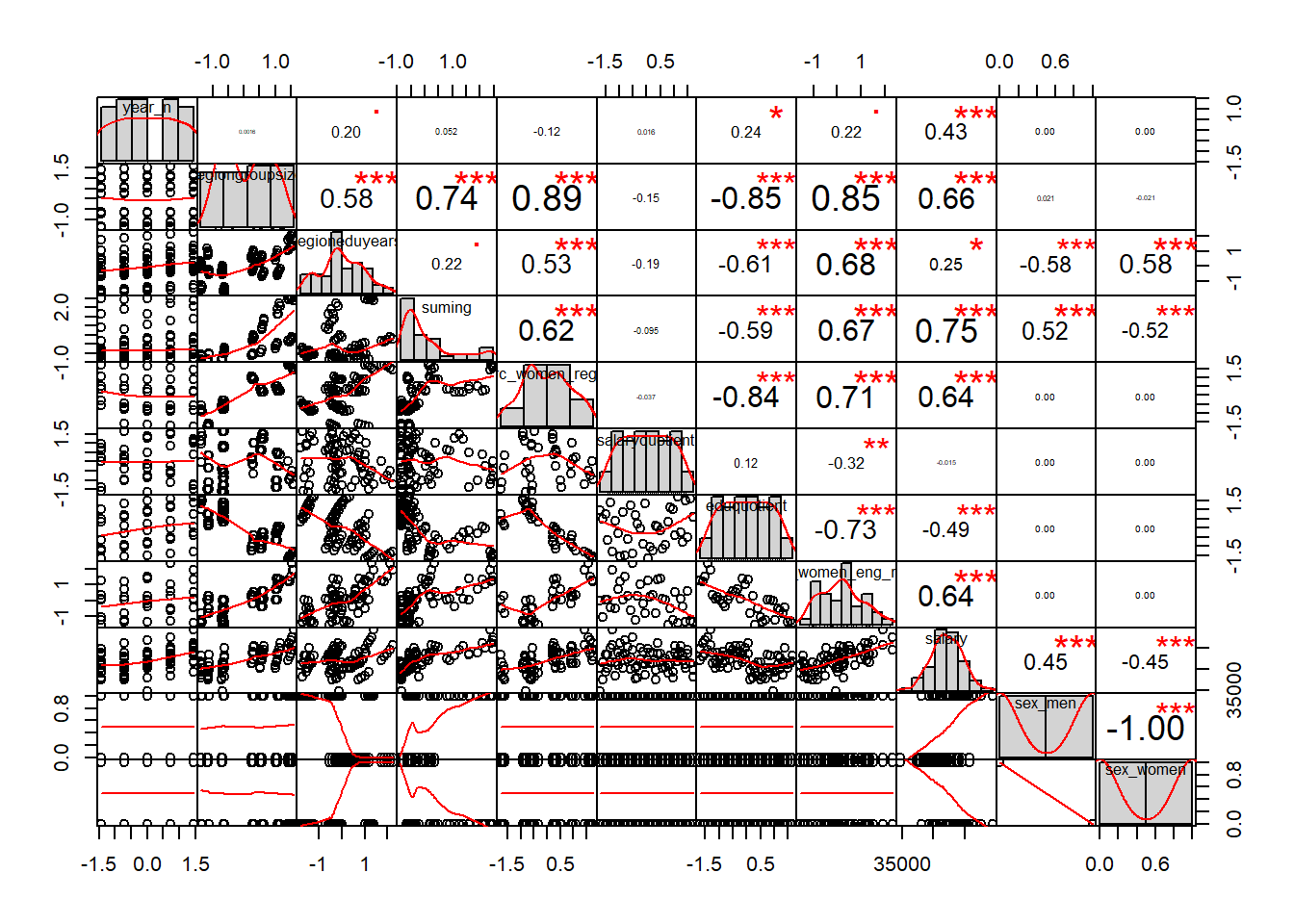Figure 1: Correlation between response and predictors and between predictors, Year 2014 - 2018 vif(tbnum) ## Warning in summary.lm(lm(object[, i] ~ object[, -i])): essentially perfect fit: ## summary may be unreliable ## Warning in summary.lm(lm(object[, i] ~ object[, -i])): essentially perfect fit: ## summary may be unreliable ## year_n regiongroupsize regioneduyears ## 4.665634 21.810910 14.478685 ## suming perc_women_region salaryquotient ## 8.688599 8.259497 1.382593 ## eduquotient perc_women_eng_region salary ## 12.400845 8.141404 8.359112 ## sex_men sex_women ## Inf Inf p1 <- tb %>% ggscatter(x = "regiongroupsize", y = "perc_women_region", add = "reg.line", conf.int = TRUE, cor.coef = TRUE, cor.method = "pearson") p2 <- tb %>% ggscatter(x = "regiongroupsize", y = "perc_women_eng_region", add = "reg.line", conf.int = TRUE, cor.coef = TRUE, cor.method = "pearson") p3 <- tb %>% ggscatter(x = "regiongroupsize", y = "eduquotient", add = "reg.line", conf.int = TRUE, cor.coef = TRUE, cor.method = "pearson") p4 <- tb %>% ggscatter(x = "perc_women_region", y = "eduquotient", add = "reg.line", conf.int = TRUE, cor.coef = TRUE, cor.method = "pearson") gridExtra::grid.arrange(p1, p2, p3, p4, ncol = 2)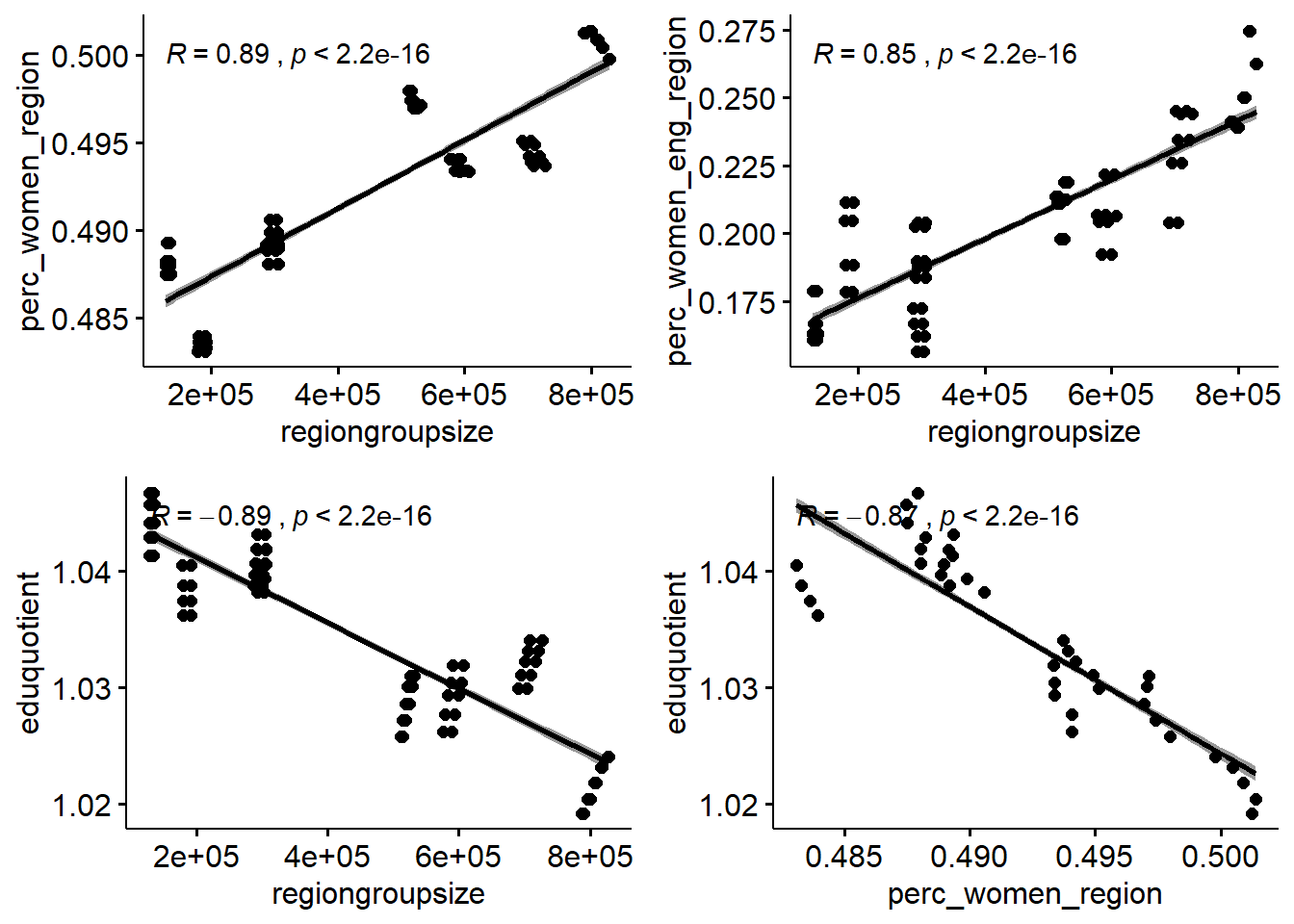Figure 2: Correlation between response and predictors and between predictors, Year 2014 - 2018 The dataset only contains 76 rows. This together with multicollinearity limits the number of predictors to include in the regression. I would like to choose the predictors that best contains most information from the dataset with respect to the response. I will use an elastic net to find the variable that contains the best signals for later use in the analysis. First I will search for the explanatory variables that best predict the response using no interactions. I will use 10-fold cross-validation with an elastic net. Elastic nets are linear and do not take into account the shape of the relations between the predictors. Alpha = 1 indicates a lasso regression. X <- model.matrix(salary ~ ., tbnum)[, -1] Y <- tbnum$salary

set.seed(123)  # for reproducibility
cv_glmnet <- train(
x = X,
y = Y,
weights = tbnum_weights,
method = "glmnet",
preProc = c("zv", "center", "scale"),
trControl = trainControl(method = "cv", number = 10),
tuneLength = 20
)
## Warning in nominalTrainWorkflow(x = x, y = y, wts = weights, info = trainInfo, :
## There were missing values in resampled performance measures.
vip(cv_glmnet, num_features = 20, geom = "point")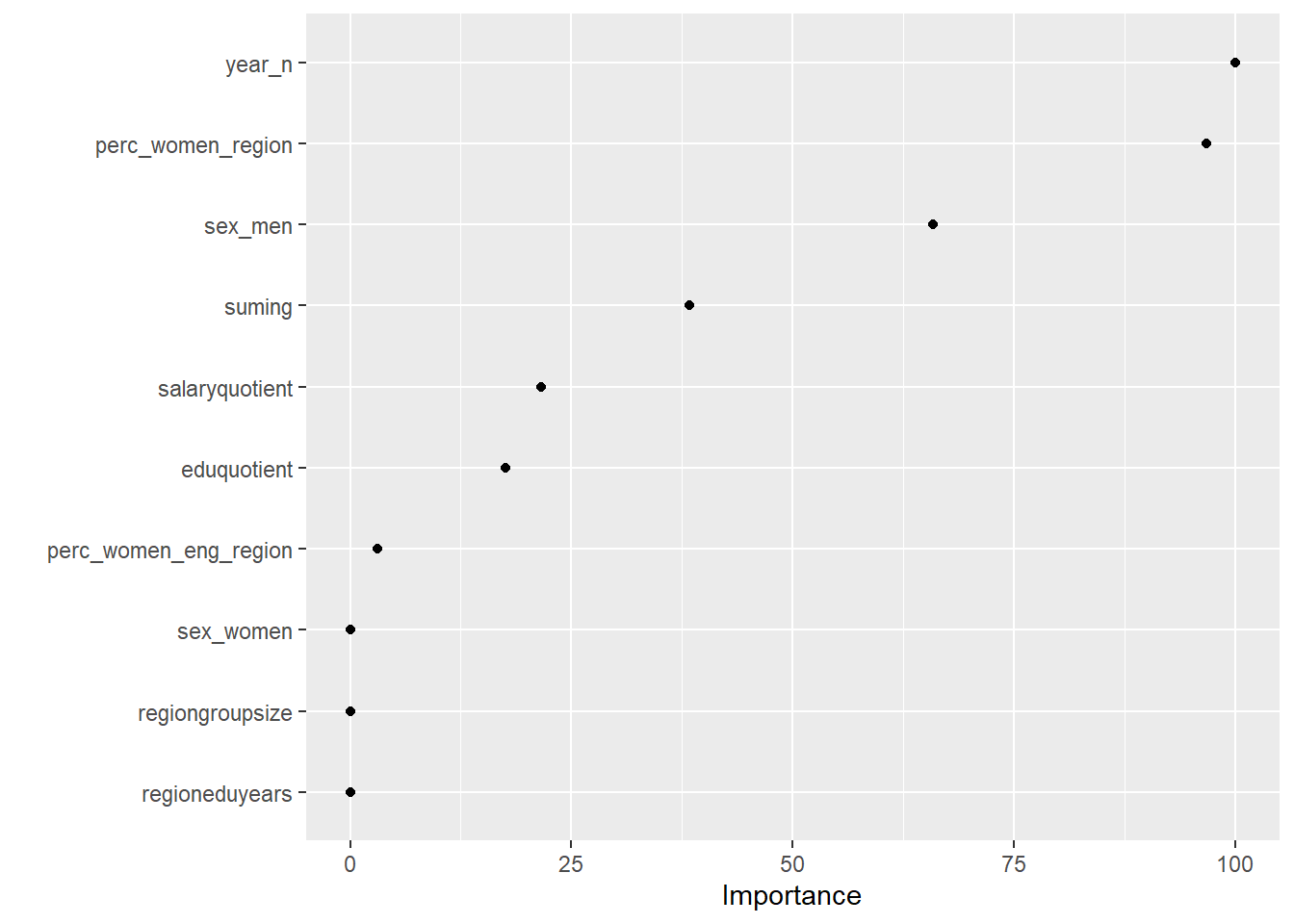Figure 3: Elastic net search on the data using no interactions, Year 2014 - 2018

cv_glmnet$bestTune ## alpha lambda ## 371 1 49.48132 elastic_min <- glmnet( x = X, y = Y, alpha = 1 ) plot(elastic_min, xvar = "lambda", main = "Elastic net penalty\n\n")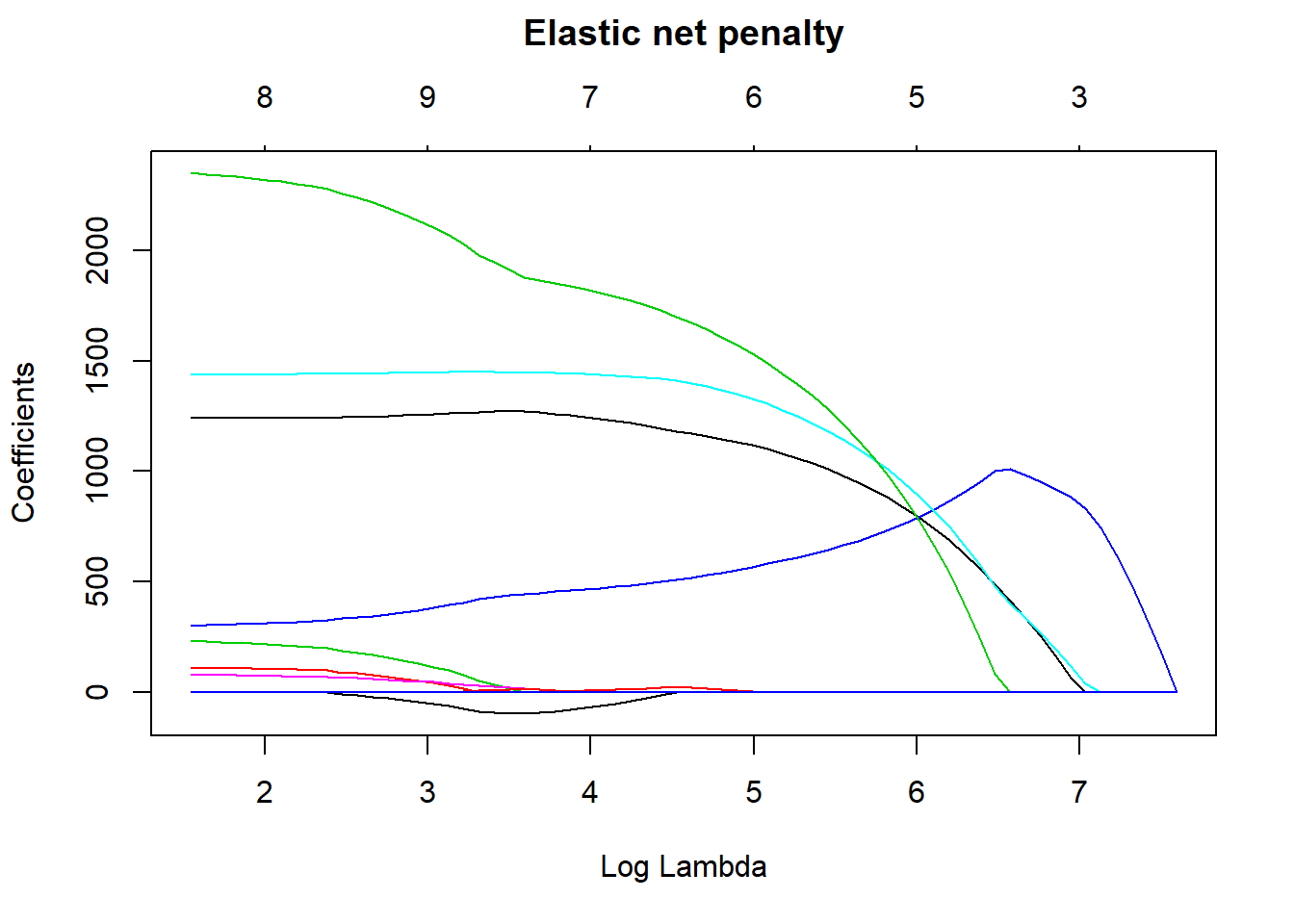Figure 4: Elastic net search on the data using no interactions, Year 2014 - 2018 Next, I will use an elastic net to find the variable that contains the best signals including interactions. temp <- dplyr::select(tbnum, -salary) f <- as.formula( ~ .*.) X <- model.matrix(f, temp)[, -1] Y <- tbnum$salary

set.seed(123)  # for reproducibility
cv_glmnet <- train(
x = X,
y = Y,
weights = tbnum_weights,
method = "glmnet",
metric = "Rsquared",
maximize = TRUE,
preProc = c("zv", "center", "scale"),
trControl = trainControl(method = "cv", number = 10),
tuneLength = 30
)
## Warning in nominalTrainWorkflow(x = x, y = y, wts = weights, info = trainInfo, :
## There were missing values in resampled performance measures.
## Warning in train.default(x = X, y = Y, weights = tbnum_weights, method =
## "glmnet", : missing values found in aggregated results
vip(cv_glmnet, num_features = 20, geom = "point")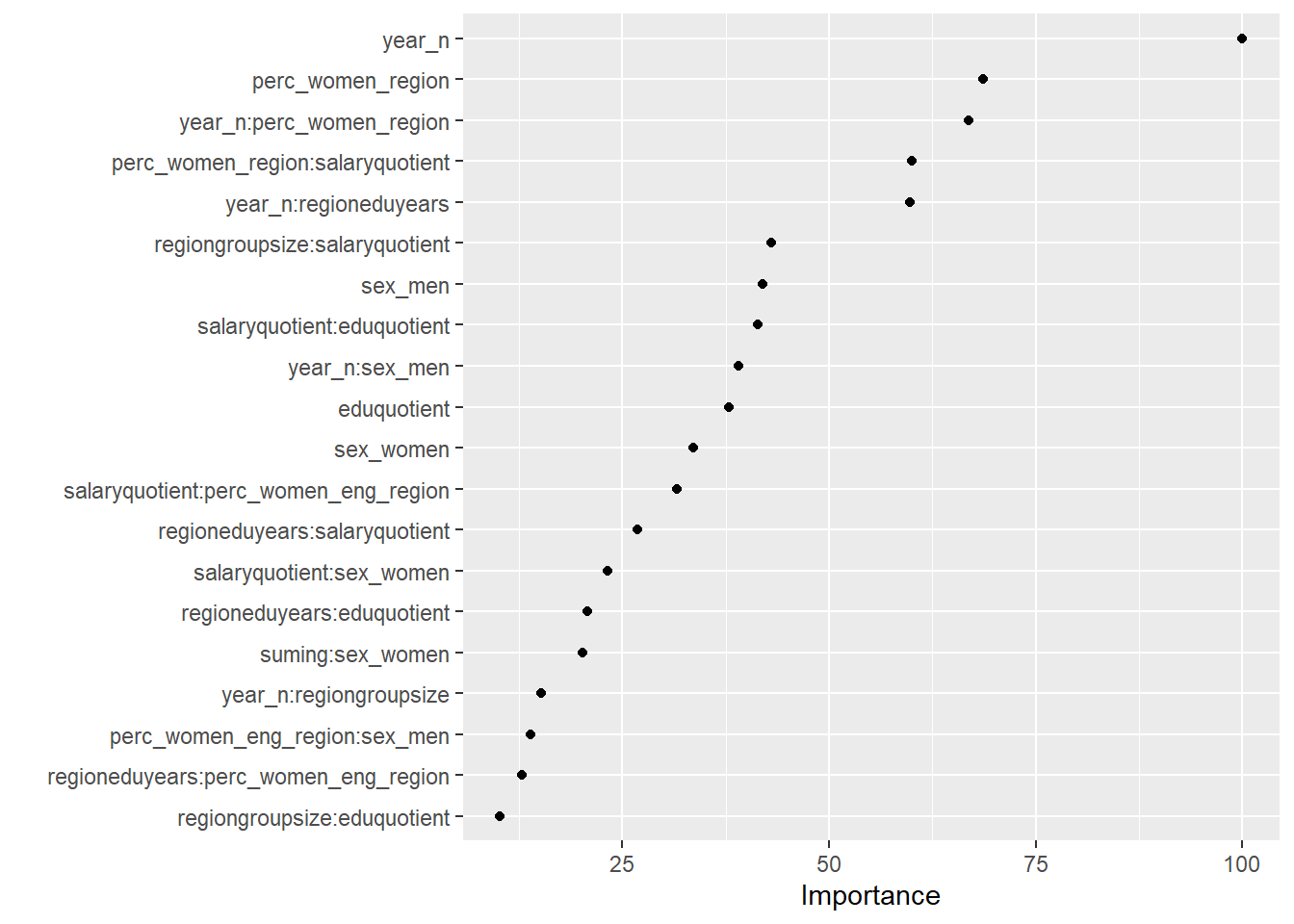Figure 5: Elastic net search on the data including interactions, Year 2014 - 2018

cv_glmnet$bestTune ## alpha lambda ## 758 0.8758621 4.280173 elastic_min <- glmnet( x = X, y = Y, alpha = 0.9 ) plot(elastic_min, xvar = "lambda", main = "Elastic net penalty\n\n")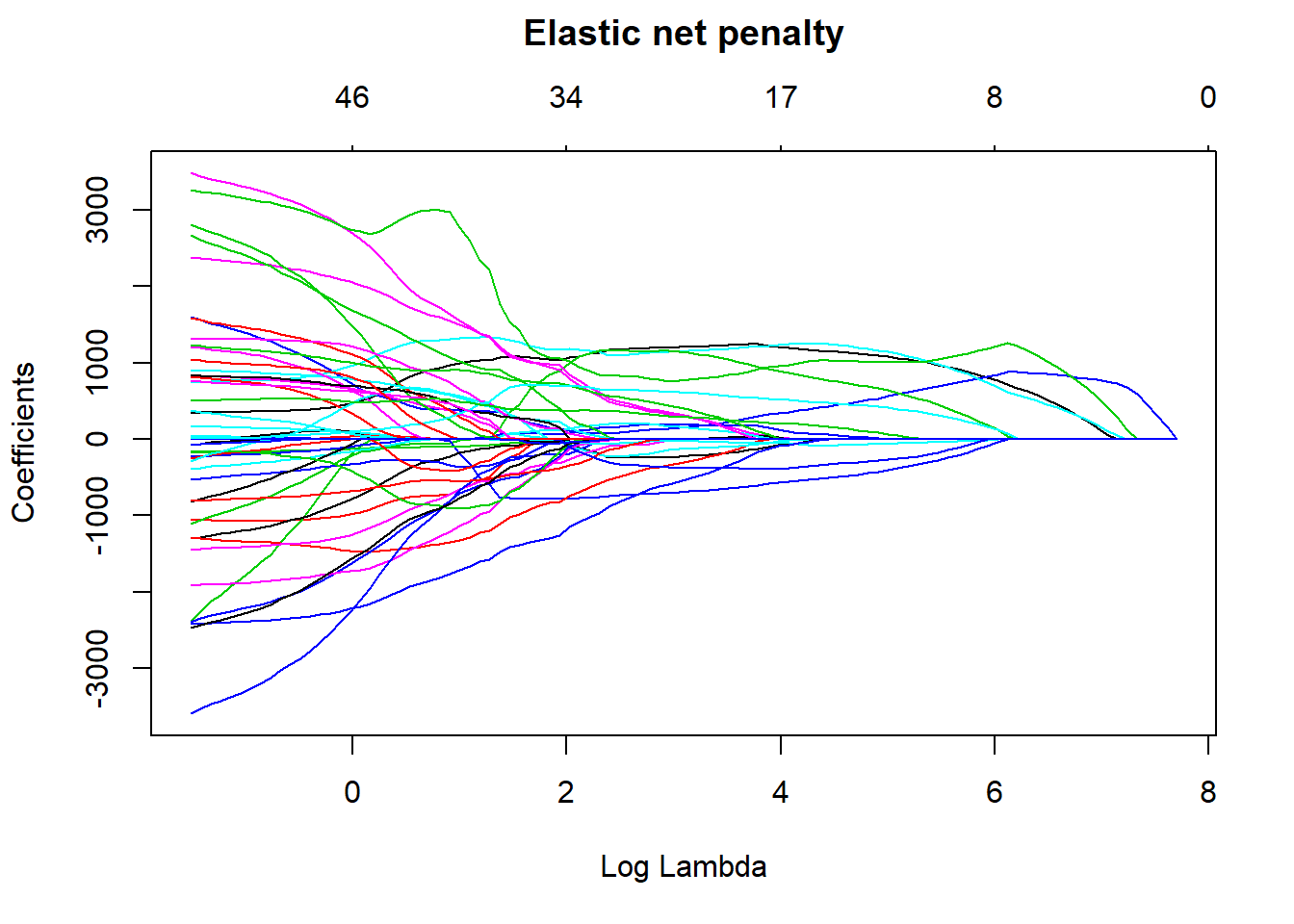Figure 6: Elastic net search on the data including interactions, Year 2014 - 2018 I use MARS to fit the best signals using from the elastic net using no interactions. Four predictors minimise the AIC while still ensuring that the coefficients are valid, testing them with bootstrap. temp <- dplyr::select(tbnum, c(salary, year_n, sex_men, perc_women_region, suming)) mmod_scaled <- earth(salary ~ ., weights = tbnum_weights, data = temp, nk = 9, degree = 1) summary (mmod_scaled) ## Call: earth(formula=salary~., data=temp, weights=tbnum_weights, degree=1, nk=9) ## ## coefficients ## (Intercept) 40889.888 ## sex_men 1684.691 ## h(0-year_n) -884.328 ## h(year_n-0) 1673.931 ## h(0.311813-perc_women_region) -1249.276 ## h(perc_women_region-0.311813) 1754.546 ## h(suming- -0.549566) 553.529 ## ## Selected 7 of 8 terms, and 4 of 4 predictors ## Termination condition: Reached nk 9 ## Importance: suming, year_n, perc_women_region, sex_men ## Weights: 21400, 6800, 11500, 3000, 2400, 500, 7000, 1900, 16000, 4100, 3... ## Number of terms at each degree of interaction: 1 6 (additive model) ## GCV 3373591806 RSS 176181393125 GRSq 0.9000639 RSq 0.9294851 plot (mmod_scaled)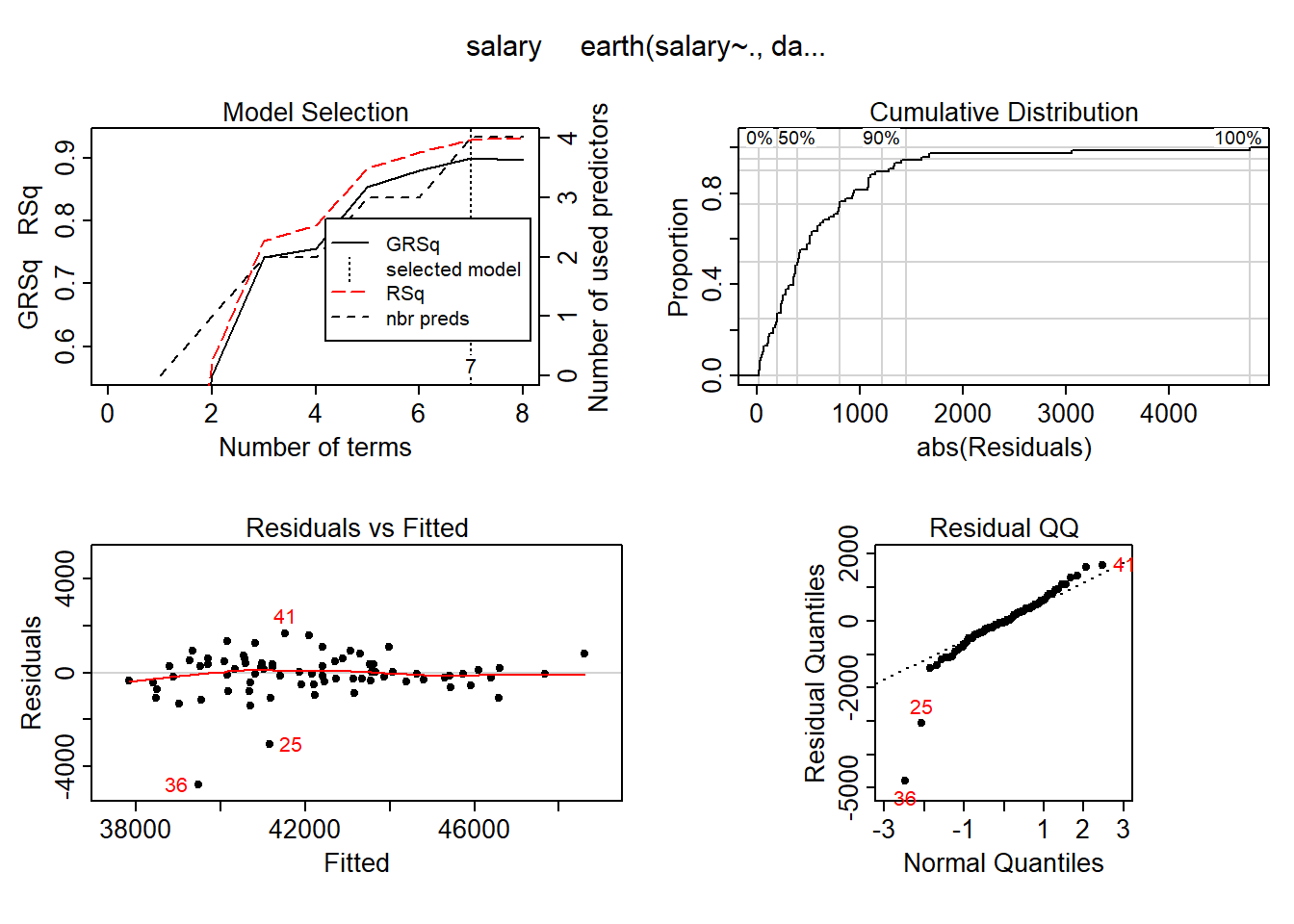Figure 7: Hockey-stick functions fit with MARS for the predictors using no interactions, Year 2014 - 2018 plotmo (mmod_scaled) ## plotmo grid: year_n sex_men perc_women_region suming ## 0 0.5 0.2069953 -0.4539968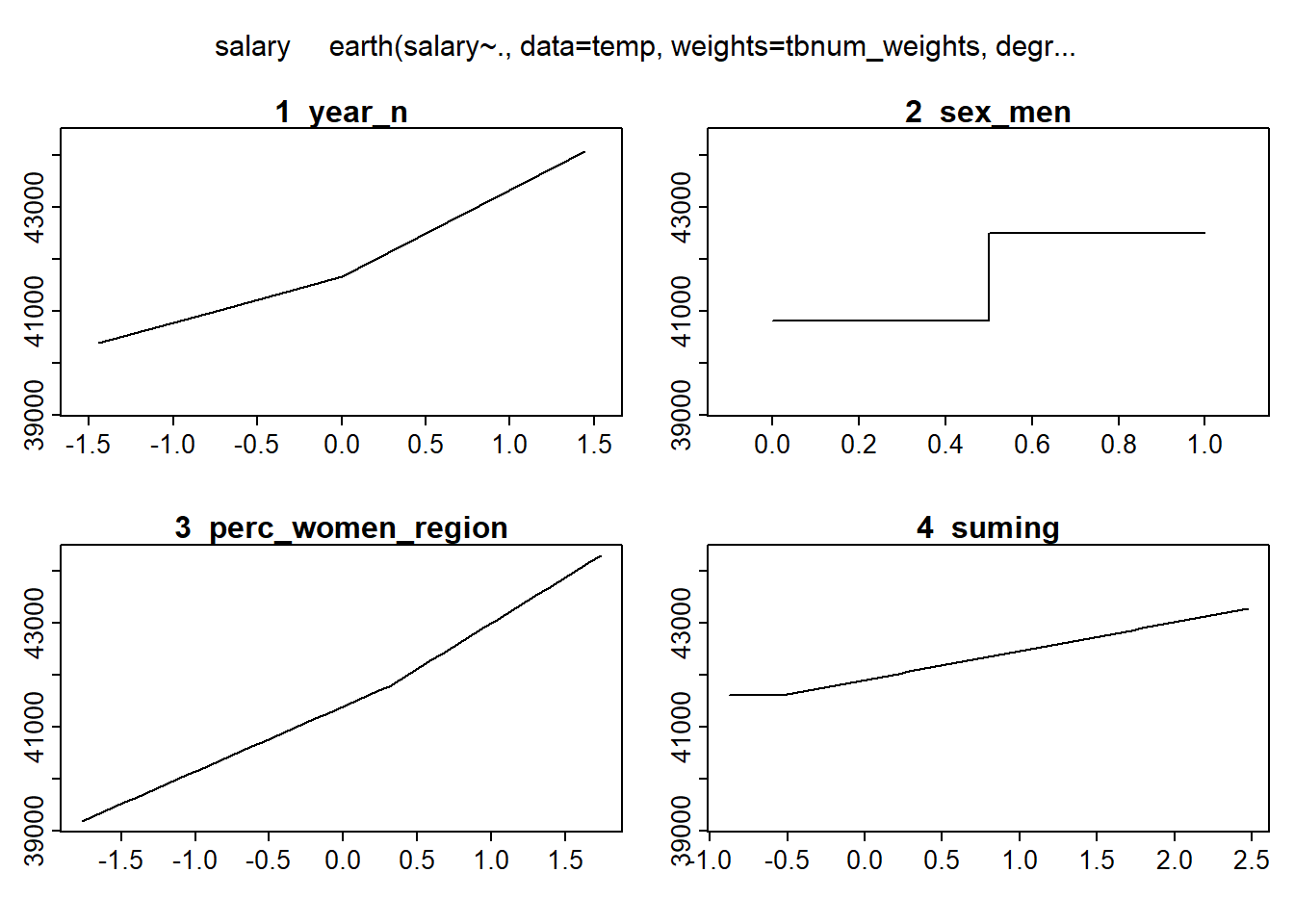Figure 8: Hockey-stick functions fit with MARS for the predictors using no interactions, Year 2014 - 2018 model_mmod_scale <- lm (salary ~ sex_men + lspline(year_n, c(0)) + lspline(perc_women_region, c(0.311813)) + lspline(suming, c(-0.549566)), weights = tbnum_weights, data = tbnum) b <- regsubsets(salary ~ sex_men + lspline(year_n, c(0)) + lspline(perc_women_region, c(0.311813)) + lspline(suming, c(-0.549566)) + lspline(suming, c(-1.22297)), data = tbnum, weights = tbnum_weights, nvmax = 12) ## Warning in leaps.setup(x, y, wt = wt, nbest = nbest, nvmax = nvmax, force.in = ## force.in, : 2 linear dependencies found ## Warning in leaps.setup(x, y, wt = wt, nbest = nbest, nvmax = nvmax, force.in = ## force.in, : nvmax reduced to 7 rs <- summary(b) AIC <- 50 * log (rs$rss / 50) + (2:8) * 2
which.min (AIC)
##  6
names (rs$which[6,])[rs$which[6,]]
##  "(Intercept)"
##  "sex_men"
##  "lspline(year_n, c(0))1"
##  "lspline(year_n, c(0))2"
##  "lspline(perc_women_region, c(0.311813))1"
##  "lspline(perc_women_region, c(0.311813))2"
##  "lspline(suming, c(-1.22297))2"
model_mmod_scale <- lm (salary ~
sex_men +
lspline(year_n, c(0)) +
lspline(perc_women_region, c(0.311813)) +
lspline(suming, c(-0.549566)),
weights = tbnum_weights,
data = tbnum)

summary (model_mmod_scale)$adj.r.squared ##  0.9244956 AIC(model_mmod_scale) ##  1258.423 set.seed(123) results <- boot(data = tbnum, statistic = bs, R = 1000, formula = as.formula(model_mmod_scale)) #conf = coefficient not passing through zero summary (model_mmod_scale) %>% tidy() %>% mutate(bootest = tidy(results)$statistic,
bootbias = tidy(results)$bias, booterr = tidy(results)$std.error,
conf = !((tidy(confint(results))$X2.5.. < 0) & (tidy(confint(results))$X97.5.. > 0)))
## Warning in norm.inter(t, adj.alpha): extreme order statistics used as endpoints
## Warning: 'tidy.matrix' is deprecated.
## See help("Deprecated")
## Warning in norm.inter(t, adj.alpha): extreme order statistics used as endpoints
## Warning: 'tidy.matrix' is deprecated.
## See help("Deprecated")
## # A tibble: 8 x 9
##   term      estimate std.error statistic  p.value bootest bootbias booterr conf
##   <chr>        <dbl>     <dbl>     <dbl>    <dbl>   <dbl>    <dbl>   <dbl> <lgl>
## 1 (Interce~   42276.     1260.     33.6  4.85e-44  42276.   -105.    1344. TRUE
## 2 sex_men      1502.      299.      5.02 4.02e- 6   1502.     27.6    428. TRUE
## 3 lspline(~     868.      164.      5.31 1.32e- 6    868.    -78.3    354. TRUE
## 4 lspline(~    1656.      159.     10.4  9.25e-16   1656.     58.4    352. TRUE
## 5 lspline(~    1049.      274.      3.82 2.88e- 4   1049.    146.     327. TRUE
## 6 lspline(~    1719.      161.     10.7  3.31e-16   1719.   -155.     265. TRUE
## 7 lspline(~    2979.     2084.      1.43 1.57e- 1   2979.   -264.    2216. FALSE
## 8 lspline(~     603.      129.      4.66 1.52e- 5    603.    -42.0    204. TRUE
plot(results, index=1) # intercept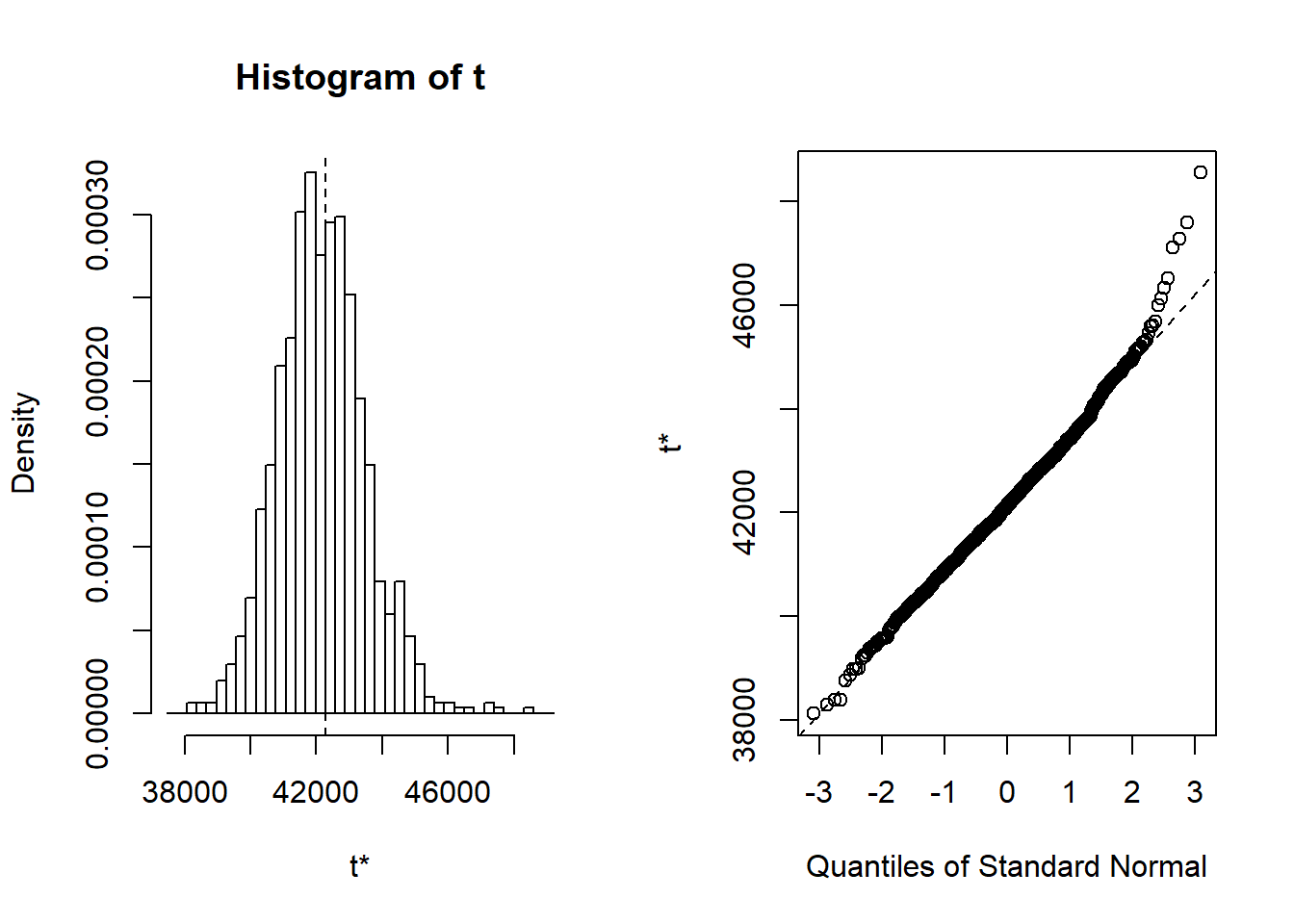Figure 9: Hockey-stick functions fit with MARS for the predictors using no interactions, Year 2014 - 2018

I will include the interaction between sex_men and salaryquotient. If I include more terms from MARS I judge that the predictions are getting unstable testing with bootstrap.

# The three best candidates from the elastic net search
model_mmod_scale <- lm (salary ~
year_n +
perc_women_region +
year_n:perc_women_region,
weights = tbnum_weights,
data = tbnum)

summary (model_mmod_scale)
##
## Call:
## lm(formula = salary ~ year_n + perc_women_region + year_n:perc_women_region,
##     data = tbnum, weights = tbnum_weights)
##
## Weighted Residuals:
##     Min      1Q  Median      3Q     Max
## -210878  -73763  -29626   45157  267181
##
## Coefficients:
##                          Estimate Std. Error t value Pr(>|t|)
## (Intercept)              42830.76     200.55 213.569  < 2e-16 ***
## year_n                    1362.05     205.00   6.644 4.97e-09 ***
## perc_women_region         1953.39     187.13  10.439 4.66e-16 ***
## year_n:perc_women_region   -32.32     194.19  -0.166    0.868
## ---
## Signif. codes:  0 '***' 0.001 '**' 0.01 '*' 0.05 '.' 0.1 ' ' 1
##
## Residual standard error: 102900 on 72 degrees of freedom
## Multiple R-squared:  0.6949, Adjusted R-squared:  0.6822
## F-statistic: 54.65 on 3 and 72 DF,  p-value: < 2.2e-16
set.seed(123)
results <- boot(data = tbnum, statistic = bs,
R = 1000, formula = as.formula(model_mmod_scale))

summary (model_mmod_scale) %>% tidy() %>%
mutate(bootest = tidy(results)$statistic, bootbias = tidy(results)$bias,
booterr =  tidy(results)$std.error, conf = !((tidy(confint(results))$X2.5.. < 0) & (tidy(confint(results))$X97.5.. > 0))) ## Warning in confint.boot(results): BCa method fails for this problem. Using ## 'perc' instead ## Warning: 'tidy.matrix' is deprecated. ## See help("Deprecated") ## Warning in confint.boot(results): BCa method fails for this problem. Using ## 'perc' instead ## Warning: 'tidy.matrix' is deprecated. ## See help("Deprecated") ## # A tibble: 4 x 9 ## term estimate std.error statistic p.value bootest bootbias booterr conf ## <chr> <dbl> <dbl> <dbl> <dbl> <dbl> <dbl> <dbl> <lgl> ## 1 (Interc~ 42831. 201. 214. 1.22e-102 42831. -776. 254. TRUE ## 2 year_n 1362. 205. 6.64 4.97e- 9 1362. 10.8 253. TRUE ## 3 perc_wo~ 1953. 187. 10.4 4.66e- 16 1953. -94.0 273. TRUE ## 4 year_n:~ -32.3 194. -0.166 8.68e- 1 -32.3 -73.3 291. FALSE temp <- dplyr::select(tbnum, c(salary, year_n, sex_men, perc_women_region, suming, salaryquotient, regioneduyears)) # A test with MARS and interactions mmod_scaled <- earth(salary ~ ., weights = tbnum_weights, data = temp, nk = 11, degree = 2) summary (mmod_scaled) ## Call: earth(formula=salary~., data=temp, weights=tbnum_weights, degree=2, ## nk=11) ## ## coefficients ## (Intercept) 41145.980 ## sex_men 1911.153 ## h(0-year_n) -959.068 ## h(year_n-0) 1703.923 ## h(0.311813-perc_women_region) -1598.885 ## h(perc_women_region-0.311813) 1546.770 ## h(suming- -0.549566) 377.482 ## sex_men * salaryquotient -526.973 ## ## Selected 8 of 9 terms, and 5 of 6 predictors ## Termination condition: Reached nk 11 ## Importance: year_n, suming, perc_women_region, sex_men, salaryquotient, ... ## Weights: 21400, 6800, 11500, 3000, 2400, 500, 7000, 1900, 16000, 4100, 3... ## Number of terms at each degree of interaction: 1 6 1 ## GCV 2668331064 RSS 116081178669 GRSq 0.9209559 RSq 0.9535396 mmod_scaled <- earth(salary ~ ., weights = tbnum_weights, data = temp, nk = 13, degree = 2) summary (mmod_scaled) ## Call: earth(formula=salary~., data=temp, weights=tbnum_weights, degree=2, ## nk=13) ## ## coefficients ## (Intercept) 41200.129 ## sex_men 1935.664 ## h(0-year_n) -867.152 ## h(year_n-0) 1727.342 ## h(0.311813-perc_women_region) -1532.402 ## h(perc_women_region-0.311813) 1450.579 ## h(suming- -0.549566) 353.205 ## h(-1.22297-salaryquotient) -3067.317 ## sex_men * salaryquotient -647.409 ## ## Selected 9 of 11 terms, and 5 of 6 predictors ## Termination condition: Reached nk 13 ## Importance: year_n, suming, perc_women_region, sex_men, salaryquotient, ... ## Weights: 21400, 6800, 11500, 3000, 2400, 500, 7000, 1900, 16000, 4100, 3... ## Number of terms at each degree of interaction: 1 7 1 ## GCV 2629100288 RSS 104645110144 GRSq 0.922118 RSq 0.9581168 plot (mmod_scaled)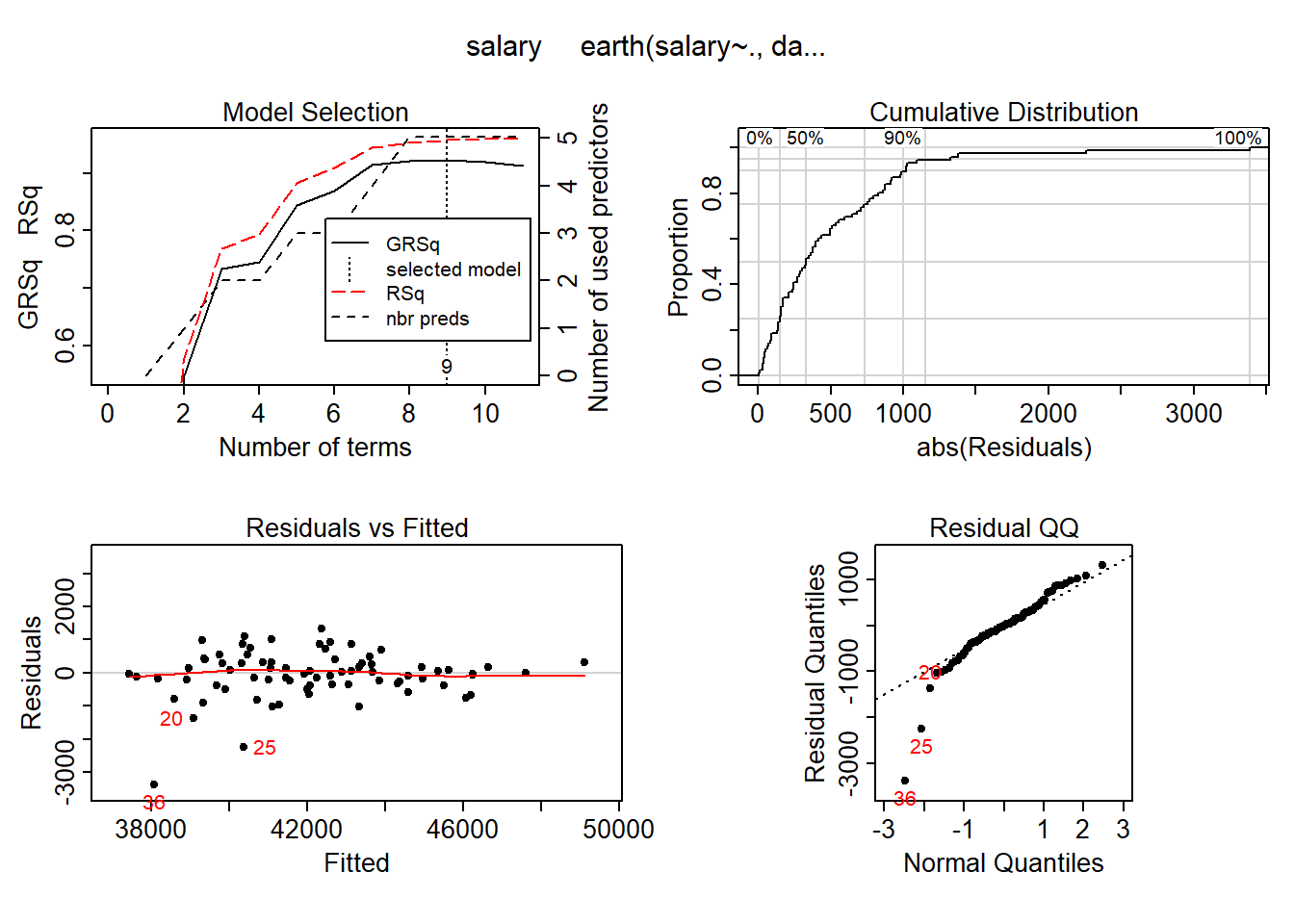Figure 10: Hockey-stick functions fit with MARS for the predictors including interactions, Year 2014 - 2018 plotmo (mmod_scaled) ## plotmo grid: year_n sex_men perc_women_region suming salaryquotient ## 0 0.5 0.2069953 -0.4539968 0 ## regioneduyears ## -0.1394781Figure 11: Hockey-stick functions fit with MARS for the predictors including interactions, Year 2014 - 2018 model_mmod_scale <- lm (salary ~ sex_men + lspline(year_n, c(0)) + lspline(perc_women_region, c(0.311813)) + lspline(suming, c(-0.549566)) + lspline(salaryquotient, c(-1.22297)) + sex_men:salaryquotient, weights = tbnum_weights, data = tbnum) summary (model_mmod_scale) ## ## Call: ## lm(formula = salary ~ sex_men + lspline(year_n, c(0)) + lspline(perc_women_region, ## c(0.311813)) + lspline(suming, c(-0.549566)) + lspline(salaryquotient, ## c(-1.22297)) + sex_men:salaryquotient, data = tbnum, weights = tbnum_weights) ## ## Weighted Residuals: ## Min 1Q Median 3Q Max ## -159343 -17893 2866 18128 79279 ## ## Coefficients: ## Estimate Std. Error t value Pr(>|t|) ## (Intercept) 44723.0 1743.3 25.655 < 2e-16 ## sex_men 1772.4 238.2 7.440 2.88e-10 ## lspline(year_n, c(0))1 868.1 131.4 6.606 8.56e-09 ## lspline(year_n, c(0))2 1710.1 122.9 13.910 < 2e-16 ## lspline(perc_women_region, c(0.311813))1 1382.3 225.1 6.142 5.51e-08 ## lspline(perc_women_region, c(0.311813))2 1518.7 139.2 10.911 2.48e-16 ## lspline(suming, c(-0.549566))1 1786.4 1624.5 1.100 0.275534 ## lspline(suming, c(-0.549566))2 395.8 105.3 3.758 0.000369 ## lspline(salaryquotient, c(-1.22297))1 2705.7 1126.2 2.403 0.019147 ## lspline(salaryquotient, c(-1.22297))2 295.7 151.4 1.953 0.055071 ## sex_men:salaryquotient -884.9 158.6 -5.578 5.07e-07 ## ## (Intercept) *** ## sex_men *** ## lspline(year_n, c(0))1 *** ## lspline(year_n, c(0))2 *** ## lspline(perc_women_region, c(0.311813))1 *** ## lspline(perc_women_region, c(0.311813))2 *** ## lspline(suming, c(-0.549566))1 ## lspline(suming, c(-0.549566))2 *** ## lspline(salaryquotient, c(-1.22297))1 * ## lspline(salaryquotient, c(-1.22297))2 . ## sex_men:salaryquotient *** ## --- ## Signif. codes: 0 '***' 0.001 '**' 0.01 '*' 0.05 '.' 0.1 ' ' 1 ## ## Residual standard error: 38700 on 65 degrees of freedom ## Multiple R-squared: 0.961, Adjusted R-squared: 0.955 ## F-statistic: 160.3 on 10 and 65 DF, p-value: < 2.2e-16 set.seed(123) results <- boot(data = tbnum, statistic = bs, R = 1000, formula = as.formula(model_mmod_scale)) summary (model_mmod_scale) %>% tidy() %>% mutate(bootest = tidy(results)$statistic,
bootbias = tidy(results)$bias, booterr = tidy(results)$std.error,
conf = !((tidy(confint(results))$X2.5.. < 0) & (tidy(confint(results))$X97.5.. > 0)))
## Warning: 'tidy.matrix' is deprecated.
## See help("Deprecated")

## Warning: 'tidy.matrix' is deprecated.
## See help("Deprecated")
## # A tibble: 11 x 9
##    term     estimate std.error statistic  p.value bootest bootbias booterr conf
##    <chr>       <dbl>     <dbl>     <dbl>    <dbl>   <dbl>    <dbl>   <dbl> <lgl>
##  1 (Interc~   44723.     1743.     25.7  1.02e-35  44723.  1007.     3439. TRUE
##  2 sex_men     1772.      238.      7.44 2.88e-10   1772.    -3.65    339. TRUE
##  3 lspline~     868.      131.      6.61 8.56e- 9    868.   -88.4     299. TRUE
##  4 lspline~    1710.      123.     13.9  3.71e-21   1710.   -11.6     250. TRUE
##  5 lspline~    1382.      225.      6.14 5.51e- 8   1382.  -104.      308. TRUE
##  6 lspline~    1519.      139.     10.9  2.48e-16   1519.   -51.2     248. TRUE
##  7 lspline~    1786.     1625.      1.10 2.76e- 1   1786.    80.7    1608. FALSE
##  8 lspline~     396.      105.      3.76 3.69e- 4    396.    47.0     171. FALSE
##  9 lspline~    2706.     1126.      2.40 1.91e- 2   2706.   904.     2568. FALSE
## 10 lspline~     296.      151.      1.95 5.51e- 2    296.    77.6     196. FALSE
## 11 sex_men~    -885.      159.     -5.58 5.07e- 7   -885.  -153.      264. TRUE

I will also use 10-fold cross-validation fit with decision trees and bagging on the data.

set.seed(123)
tbnum_bag <- train(
salary ~ .,
data = tbnum,
method = "treebag",
weights = tbnum_weights,
trControl = trainControl(method = "cv", number = 10),
nbagg = 200,
control = rpart.control(minsplit = 2, cp = 0)
)

vip::vip(tbnum_bag, num_features = 20, bar = FALSE)
## Warning in vip.default(tbnum_bag, num_features = 20, bar = FALSE): The bar
## argument has been deprecated in favor of the new geom argument. It will be
## removed in version 0.3.0.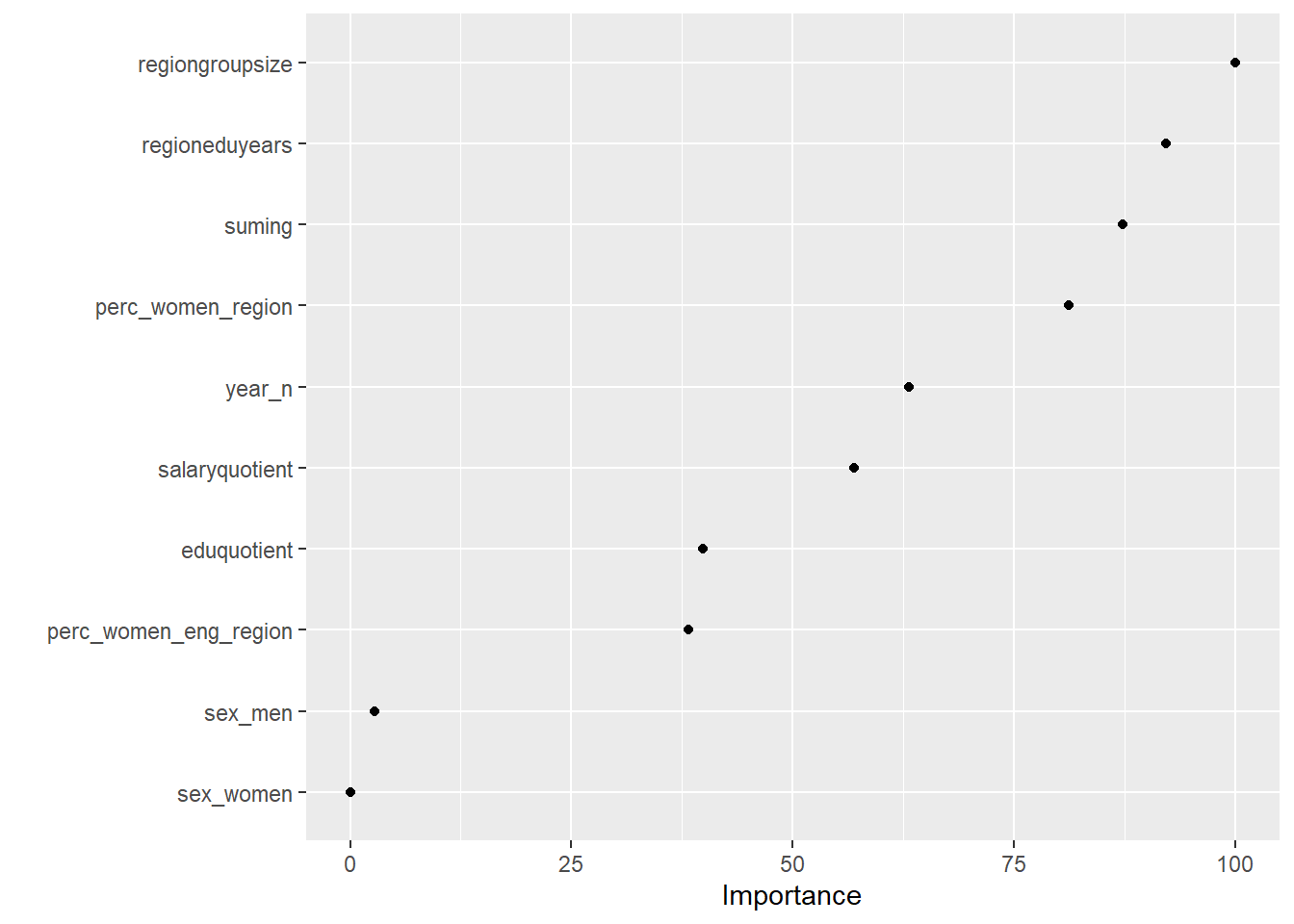Figure 12: Data fit with decision tree bag, Year 2014 - 2018

Perform diagnostics on the final model.

model <- lm (salary ~
sex_men +
lspline(year_n, c(0)) +
lspline(perc_women_region, c(0.311813)) +
lspline(suming, c(-0.549566)) +
sex_men:salaryquotient,
weights = tbnum_weights,
data = tbnum)

summary (model)
##
## Call:
## lm(formula = salary ~ sex_men + lspline(year_n, c(0)) + lspline(perc_women_region,
##     c(0.311813)) + lspline(suming, c(-0.549566)) + sex_men:salaryquotient,
##     data = tbnum, weights = tbnum_weights)
##
## Weighted Residuals:
##     Min      1Q  Median      3Q     Max
## -158211  -13213   -2260   20760   84251
##
## Coefficients:
##                                          Estimate Std. Error t value Pr(>|t|)
## (Intercept)                              41612.74    1045.30  39.809  < 2e-16
## sex_men                                   1806.47     252.49   7.155 8.00e-10
## lspline(year_n, c(0))1                     948.66     135.66   6.993 1.56e-09
## lspline(year_n, c(0))2                    1693.55     131.00  12.928  < 2e-16
## lspline(perc_women_region, c(0.311813))1  1481.96     238.41   6.216 3.72e-08
## lspline(perc_women_region, c(0.311813))2  1531.75     136.49  11.222  < 2e-16
## lspline(suming, c(-0.549566))1            1625.10    1734.28   0.937  0.35210
## lspline(suming, c(-0.549566))2             408.52     112.02   3.647  0.00052
## sex_men:salaryquotient                    -515.55      89.72  -5.746 2.44e-07
##
## (Intercept)                              ***
## sex_men                                  ***
## lspline(year_n, c(0))1                   ***
## lspline(year_n, c(0))2                   ***
## lspline(perc_women_region, c(0.311813))1 ***
## lspline(perc_women_region, c(0.311813))2 ***
## lspline(suming, c(-0.549566))1
## lspline(suming, c(-0.549566))2           ***
## sex_men:salaryquotient                   ***
## ---
## Signif. codes:  0 '***' 0.001 '**' 0.01 '*' 0.05 '.' 0.1 ' ' 1
##
## Residual standard error: 41350 on 67 degrees of freedom
## Multiple R-squared:  0.9541, Adjusted R-squared:  0.9487
## F-statistic: 174.2 on 8 and 67 DF,  p-value: < 2.2e-16
anova (model)
## Analysis of Variance Table
##
## Response: salary
##                                         Df     Sum Sq    Mean Sq F value
## sex_men                                  1 4.1914e+11 4.1914e+11 245.090
## lspline(year_n, c(0))                    2 6.3234e+11 3.1617e+11 184.879
## lspline(perc_women_region, c(0.311813))  2 1.2213e+12 6.1063e+11 357.064
## lspline(suming, c(-0.549566))            2 5.4719e+10 2.7360e+10  15.998
## sex_men:salaryquotient                   1 5.6461e+10 5.6461e+10  33.015
## Residuals                               67 1.1458e+11 1.7101e+09
##                                            Pr(>F)
## sex_men                                 < 2.2e-16 ***
## lspline(year_n, c(0))                   < 2.2e-16 ***
## lspline(perc_women_region, c(0.311813)) < 2.2e-16 ***
## lspline(suming, c(-0.549566))           2.090e-06 ***
## sex_men:salaryquotient                  2.439e-07 ***
## Residuals
## ---
## Signif. codes:  0 '***' 0.001 '**' 0.01 '*' 0.05 '.' 0.1 ' ' 1
plot (model)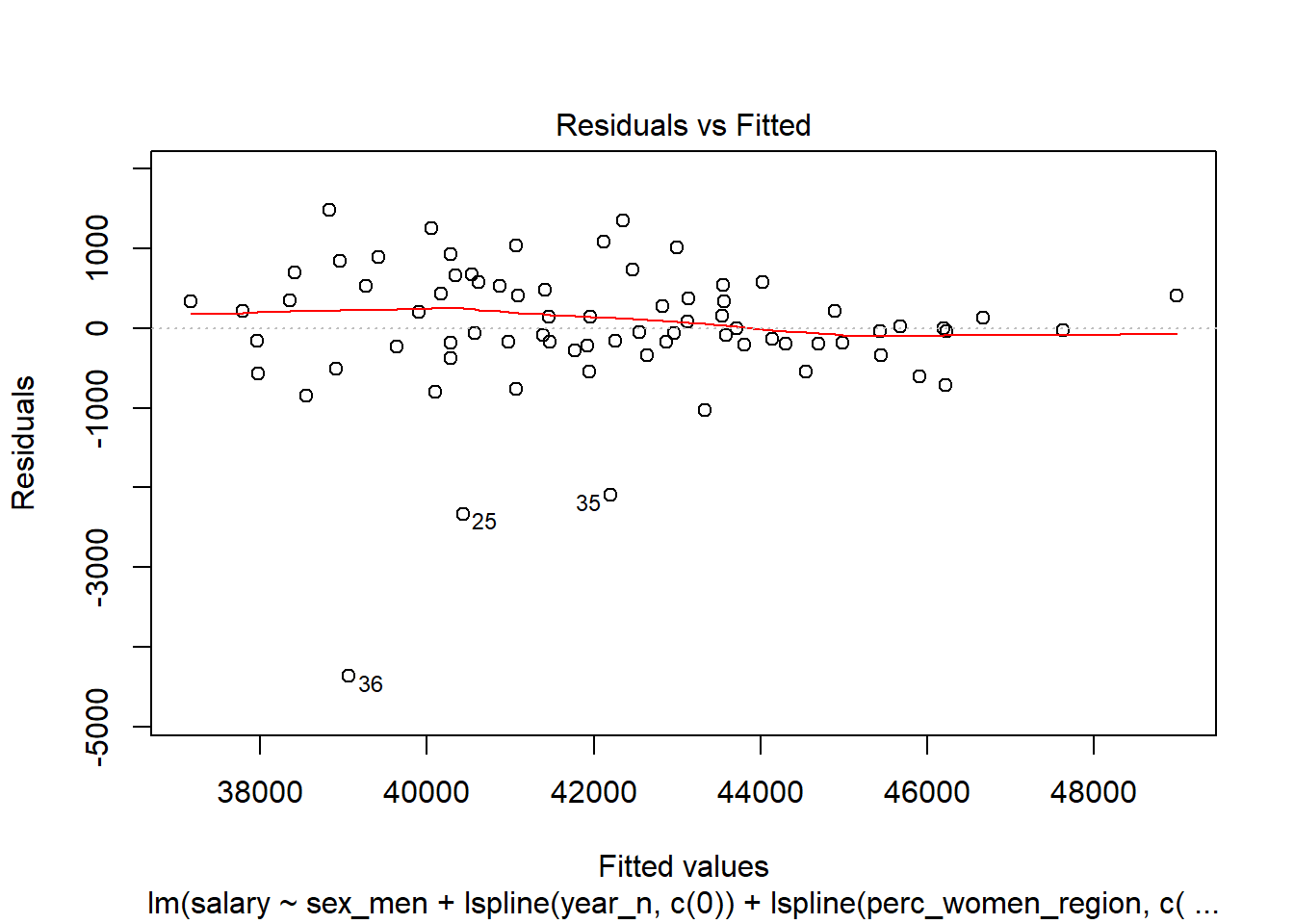Figure 13: Diagnostics on the model, Year 2014 - 2018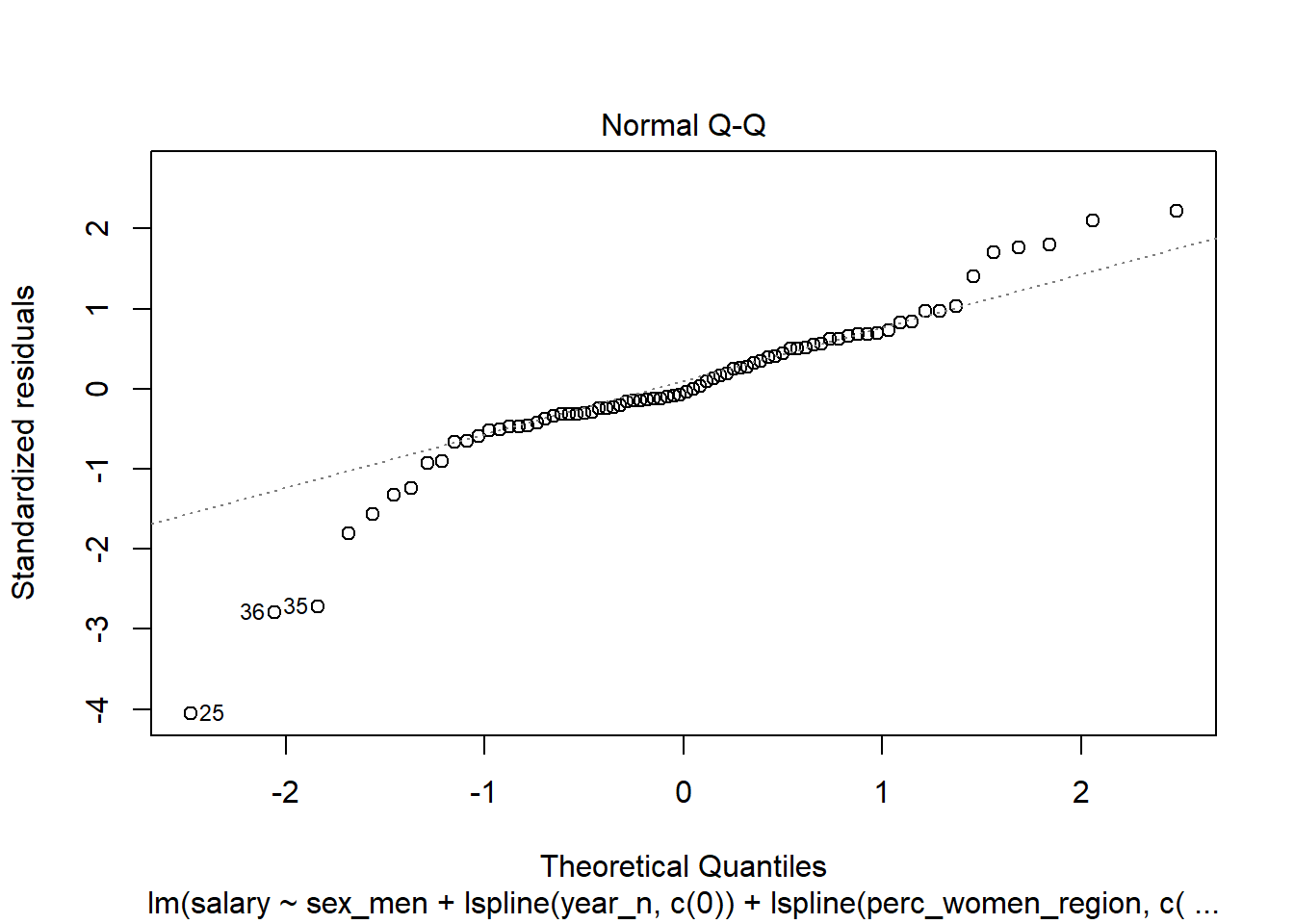Figure 14: Diagnostics on the model, Year 2014 - 2018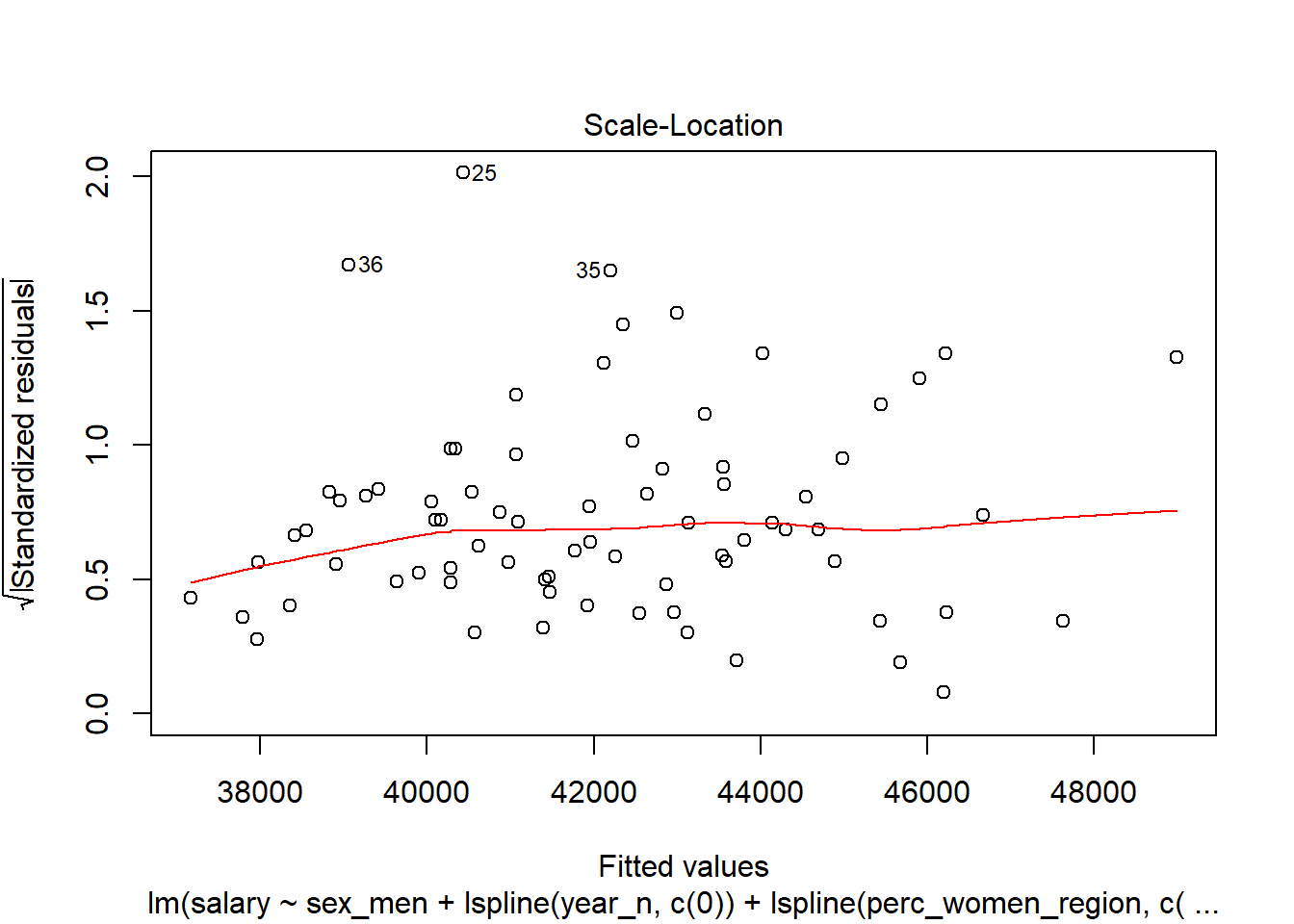Figure 15: Diagnostics on the model, Year 2014 - 2018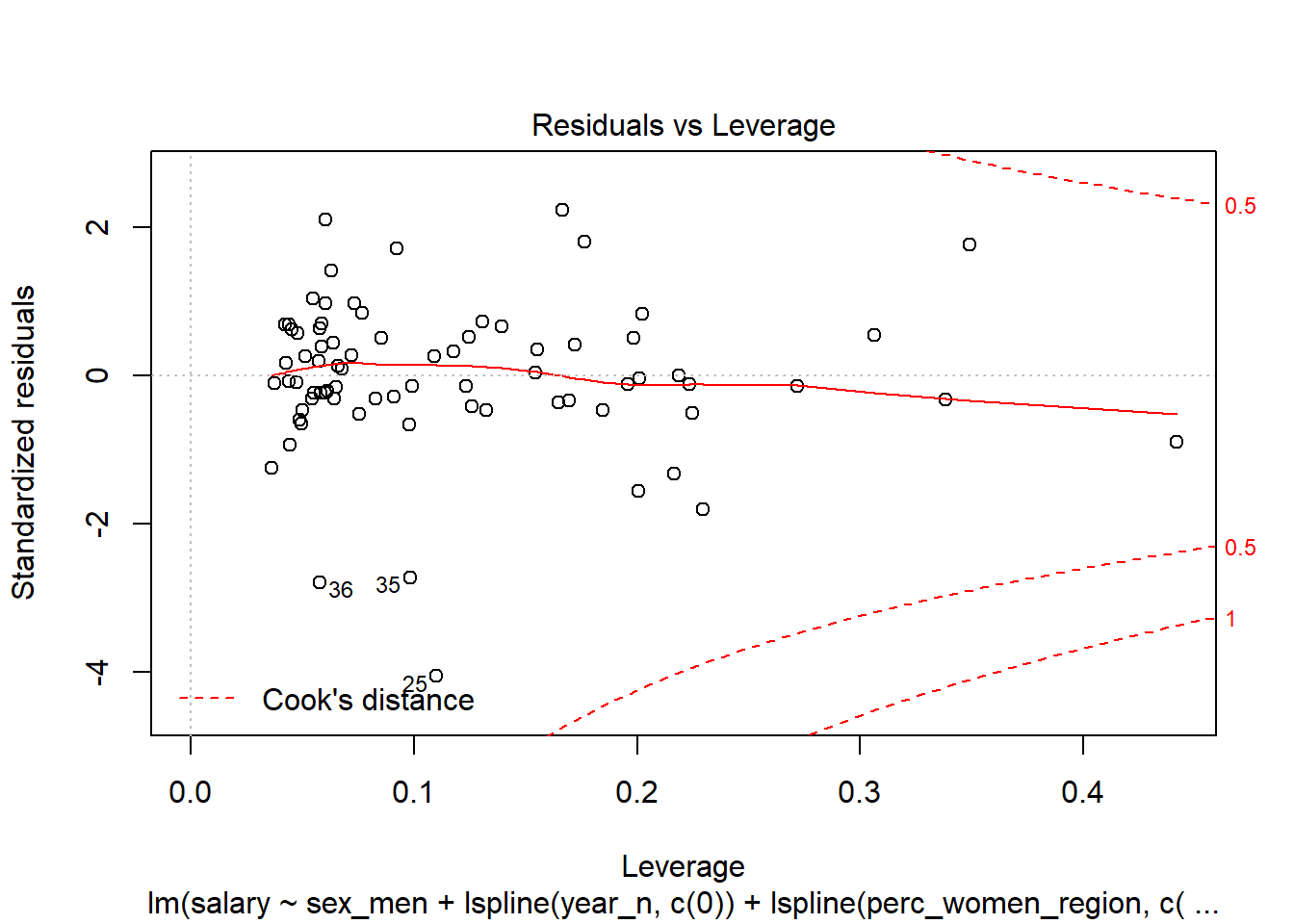Figure 16: Diagnostics on the model, Year 2014 - 2018

binnedplot(predict(model), resid(model))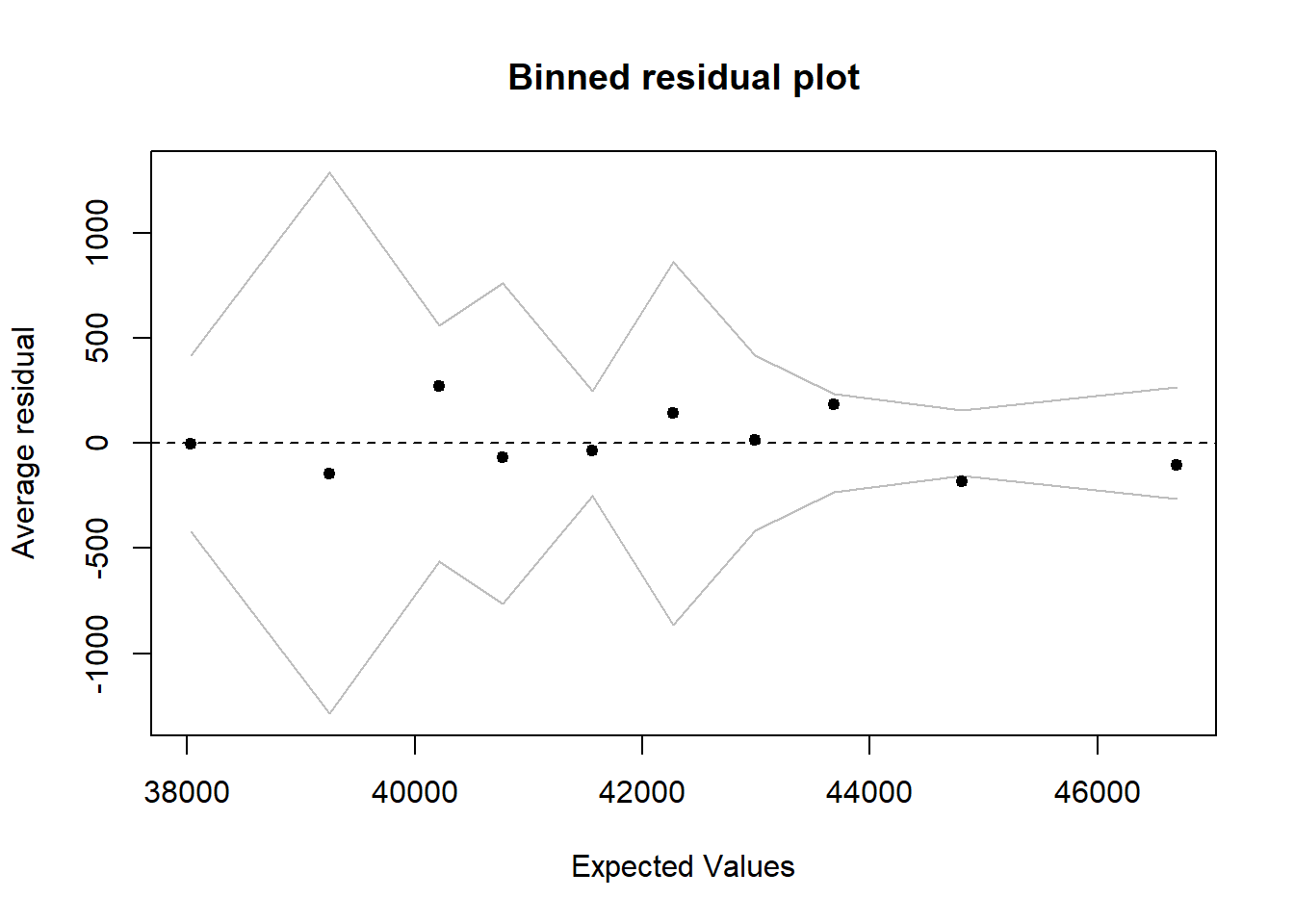Figure 17: Diagnostics on the model, Year 2014 - 2018

halfnorm(rstudent(model))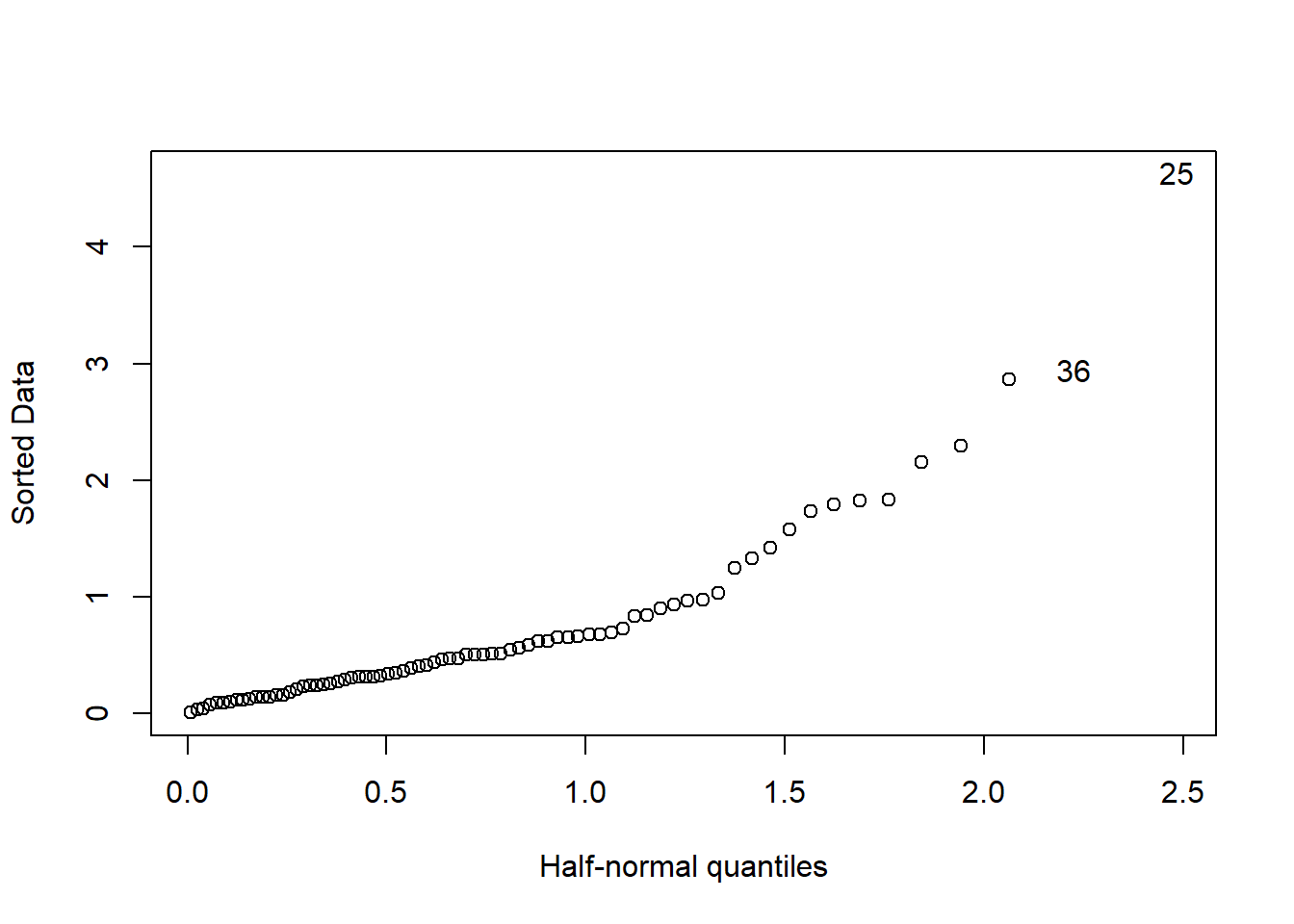Figure 18: Diagnostics on the model, Year 2014 - 2018

tbnum %>% mutate(residuals = residuals(model)) %>%
group_by(salary, perc_women_region, suming, year_n, sex_men) %>%
summarise(residuals = mean(residuals), count = sum(suming)) %>%
ggplot (aes(x = salary, y = residuals, size = sqrt(count), colour = perc_women_region)) +
geom_point() + facet_grid(. ~ year_n)
## Warning in sqrt(count): NaNs produced

## Warning in sqrt(count): NaNs produced
## Warning: Removed 49 rows containing missing values (geom_point).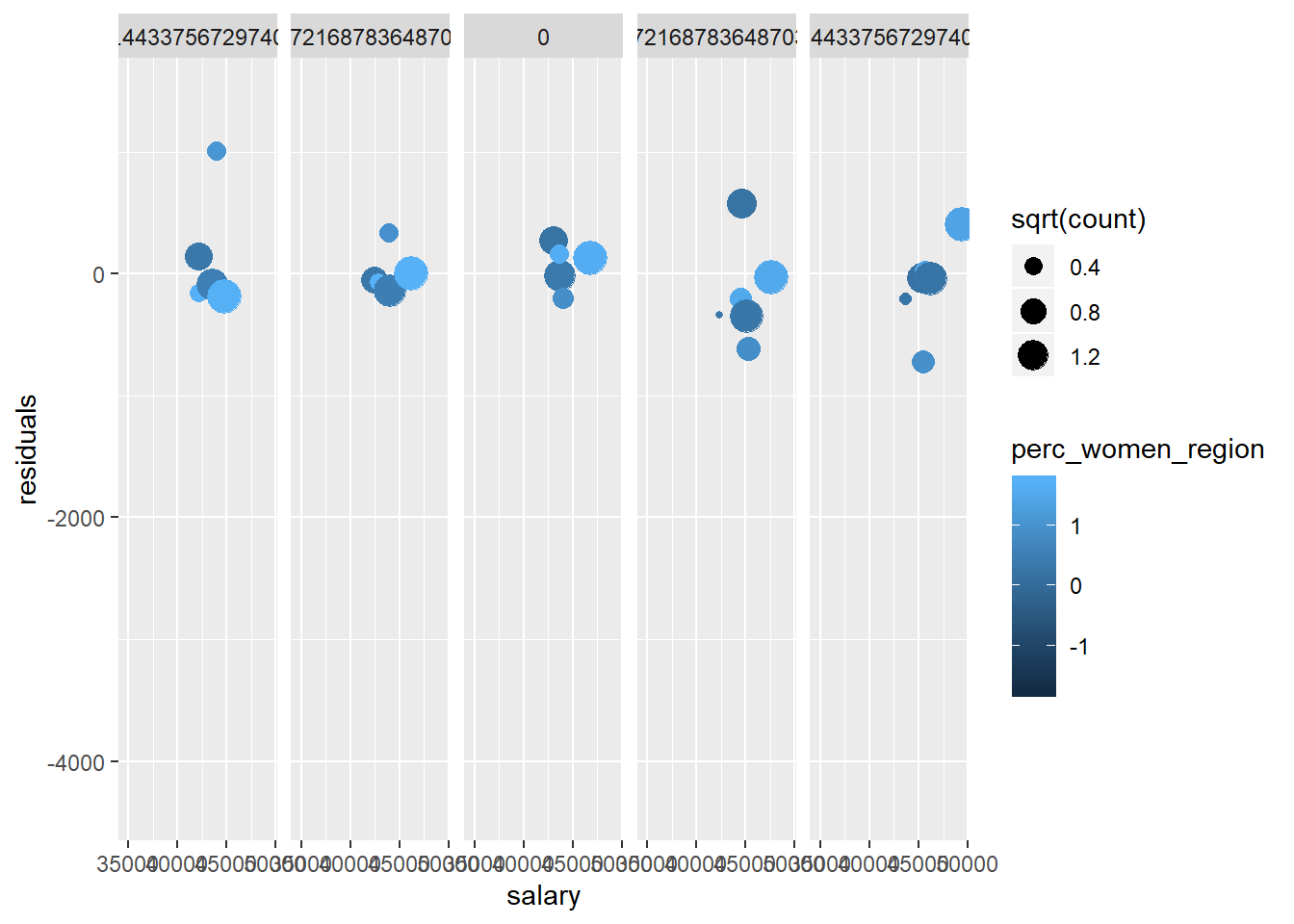Figure 19: Diagnostics on the model, Year 2014 - 2018

set.seed(123)
results <- boot(data = tbnum, statistic = bs,
R = 1000, formula = as.formula(model))

summary (model) %>% tidy() %>%
mutate(bootest = tidy(results)$statistic, bootbias = tidy(results)$bias,
booterr =  tidy(results)$std.error, conf = !((tidy(confint(results))$X2.5.. < 0) & (tidy(confint(results))\$X97.5.. > 0)))
## Warning: 'tidy.matrix' is deprecated.
## See help("Deprecated")
## Warning: 'tidy.matrix' is deprecated.
## See help("Deprecated")
## # A tibble: 9 x 9
##   term      estimate std.error statistic  p.value bootest bootbias booterr conf
##   <chr>        <dbl>     <dbl>     <dbl>    <dbl>   <dbl>    <dbl>   <dbl> <lgl>
## 1 (Interce~   41613.    1045.     39.8   2.33e-48  41613.    50.1    1258. TRUE
## 2 sex_men      1806.     252.      7.15  8.00e-10   1806.    -5.79    402. TRUE
## 3 lspline(~     949.     136.      6.99  1.56e- 9    949.   -99.1     358. TRUE
## 4 lspline(~    1694.     131.     12.9   7.16e-20   1694.    26.7     329. TRUE
## 5 lspline(~    1482.     238.      6.22  3.72e- 8   1482.    10.7     326. TRUE
## 6 lspline(~    1532.     136.     11.2   4.88e-17   1532.  -119.      241. TRUE
## 7 lspline(~    1625.    1734.      0.937 3.52e- 1   1625.   118.     2169. FALSE
## 8 lspline(~     409.     112.      3.65  5.20e- 4    409.    19.5     196. TRUE
## 9 sex_men:~    -516.      89.7    -5.75  2.44e- 7   -516.    12.3     246. TRUE
plot(results, index = 1) # intercept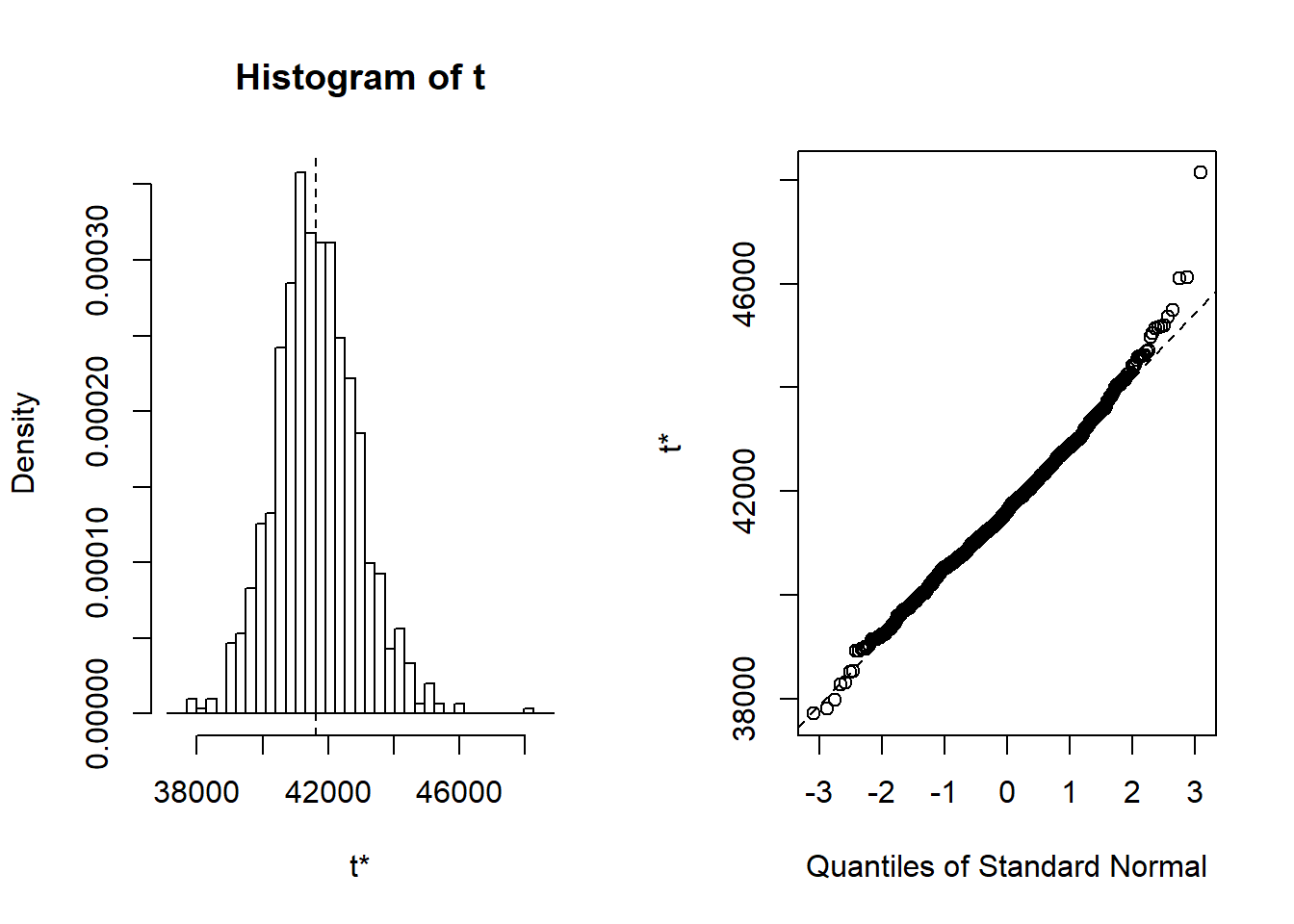Figure 20: Diagnostics on the model, Year 2014 - 2018

Let’s have a look at the outliers.

tb_outliers_info[25,]
## # A tibble: 1 x 11
##   region salary year_n regiongroupsize sex   regioneduyears suming
##   <chr>   <dbl>  <dbl>           <dbl> <chr>          <dbl>  <dbl>
## 1 SE31 ~  38100   2015          304987 men             11.2   4600
## # ... with 4 more variables: perc_women_region <dbl>, salaryquotient <dbl>,
## #   eduquotient <dbl>, perc_women_eng_region <dbl>
tb_outliers_info[35,]
## # A tibble: 1 x 11
##   region salary year_n regiongroupsize sex   regioneduyears suming
##   <chr>   <dbl>  <dbl>           <dbl> <chr>          <dbl>  <dbl>
## 1 SE21 ~  40100   2016          304366 men             11.3   2600
## # ... with 4 more variables: perc_women_region <dbl>, salaryquotient <dbl>,
## #   eduquotient <dbl>, perc_women_eng_region <dbl>
tb_outliers_info[36,]
## # A tibble: 1 x 11
##   region salary year_n regiongroupsize sex   regioneduyears suming
##   <chr>   <dbl>  <dbl>           <dbl> <chr>          <dbl>  <dbl>
## 1 SE21 ~  34700   2016          290140 women           11.7    660
## # ... with 4 more variables: perc_women_region <dbl>, salaryquotient <dbl>,
## #   eduquotient <dbl>, perc_women_eng_region <dbl>

Now let’s see what we have found. I will plot both the regression and the decision trees models for comparison.

temp <- dplyr::select(tb_unique, c(salary, year_n, sex, perc_women_region, suming, salaryquotient, regioneduyears))

mmod <- earth(salary ~ ., weights = tbnum_weights, data = temp, nk = 11, degree = 2)

summary(mmod)
## Call: earth(formula=salary~., data=temp, weights=tbnum_weights, degree=2,
##             nk=11)
##
##                               coefficients
## (Intercept)                      43393.134
## sexwomen                         -1907.433
## h(2016-year_n)                    -704.320
## h(year_n-2016)                    1184.617
## h(0.493906-perc_women_region)  -250482.793
## h(perc_women_region-0.493906)   295538.165
## h(suming-2400)                       0.067
## h(0.925101-salaryquotient)      -31088.734
## h(salaryquotient-0.925101)      -18920.288
##
## Selected 9 of 10 terms, and 5 of 6 predictors
## Termination condition: Reached nk 11
## Importance: year_n, suming, perc_women_region, sexwomen, salaryquotient, ...
## Weights: 21400, 6800, 11500, 3000, 2400, 500, 7000, 1900, 16000, 4100, 3...
## Number of terms at each degree of interaction: 1 8 (additive model)
## GCV 3188847459    RSS 126924520583    GRSq 0.9055366    RSq 0.9491997
model <- lm (salary ~
sex +
lspline(year_n, c(2016)) +
lspline(perc_women_region, c(0.493906)) +
lspline(suming, c(2400)) +
sex:salaryquotient,
weights = tbnum_weights,
data = tb_unique)

set.seed(123)  # for reproducibility
tbnum_bag <- train(
salary ~ .,
data = tb_unique,
method = "treebag",
weights = suming,
trControl = trainControl(method = "cv", number = 10),
nbagg = 200,
control = rpart.control(minsplit = 2, cp = 0)
)

p1 <- plot_model (model, type = "pred", terms = c("perc_women_region"))

p2 <- partial(tbnum_bag, pred.var = "perc_women_region") %>% autoplot()

gridExtra::grid.arrange(p1, p2, ncol = 2)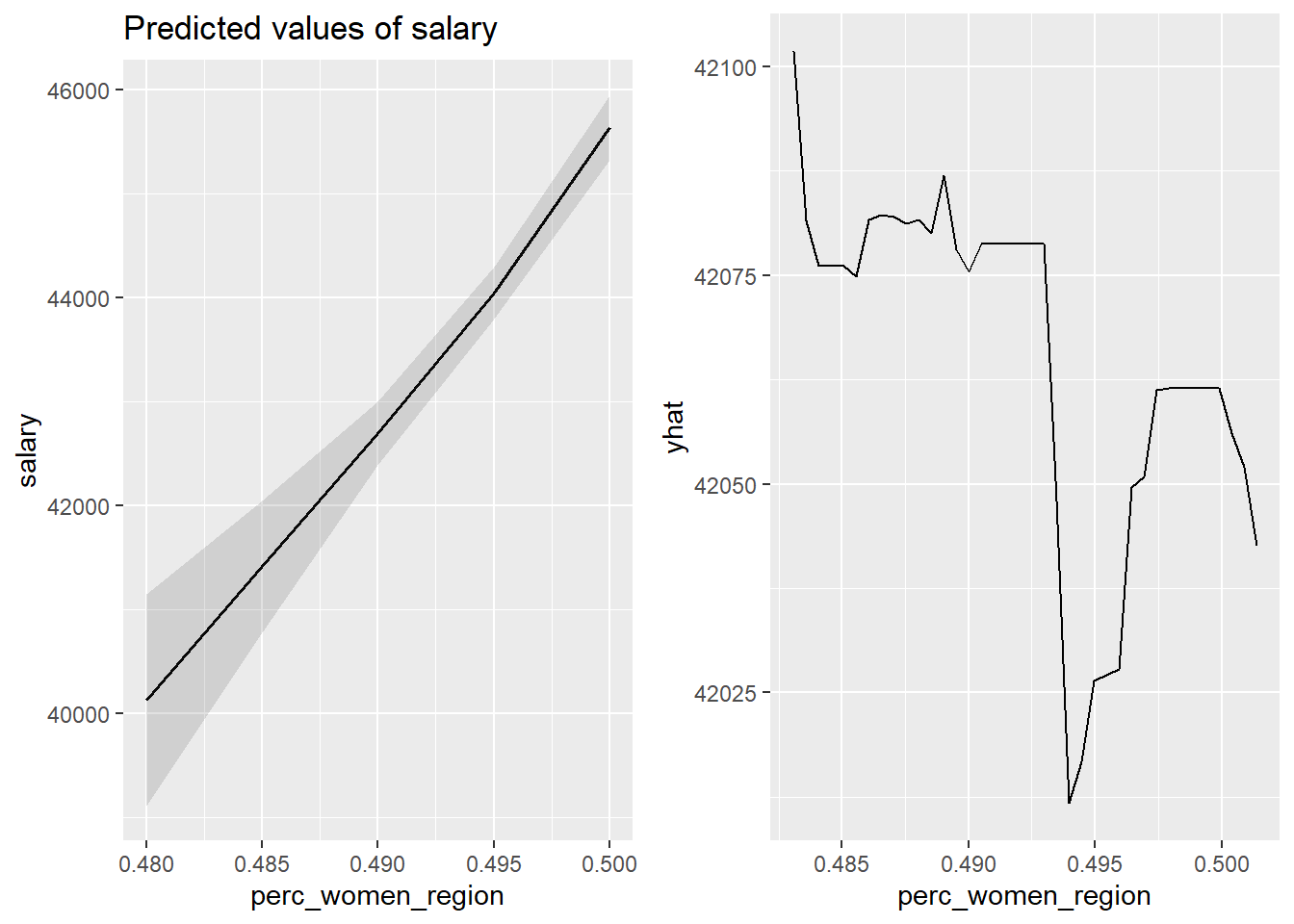Figure 21: The significance of the per cent women in the region on the salary for engineers, Year 2014 - 2018

tb_unique %>%
ggplot () +
geom_jitter (mapping = aes(x = perc_women_region, y = salary)) +
labs(
x = "Percent women in region",
y = "Salary"
)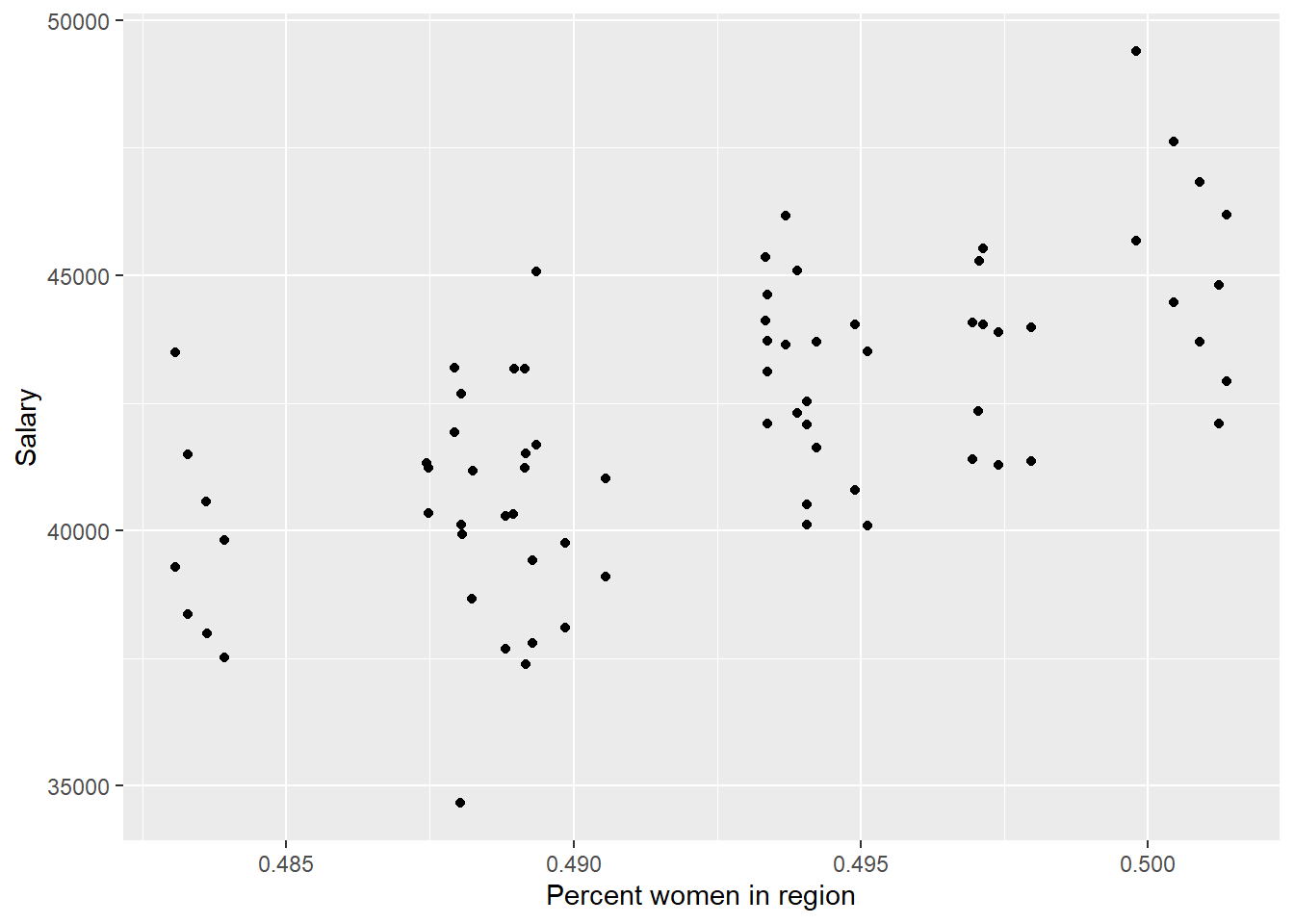Figure 22: The significance of the per cent women in the region on the salary for engineers, Year 2014 - 2018

p1 <- plot_model (model, type = "pred", terms = c("year_n"))

p2 <- partial(tbnum_bag, pred.var = "year_n") %>% autoplot()

gridExtra::grid.arrange(p1, p2, ncol = 2)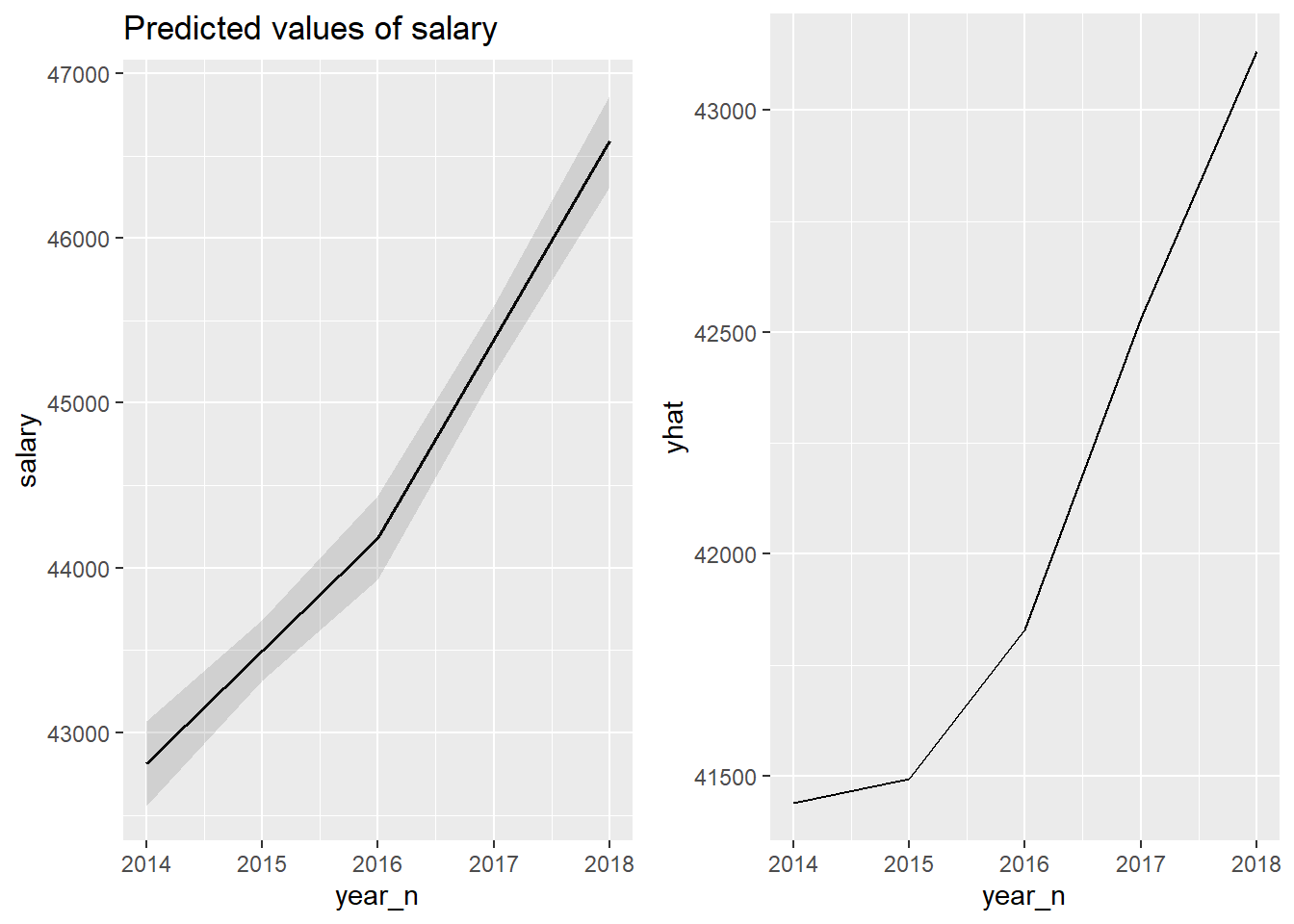Figure 23: The significance of the year on the salary for engineers, Year 2014 - 2018

tb_unique %>%
ggplot () +
geom_jitter (mapping = aes(x = year_n, y = salary)) +
labs(
x = "Year",
y = "Salary"
)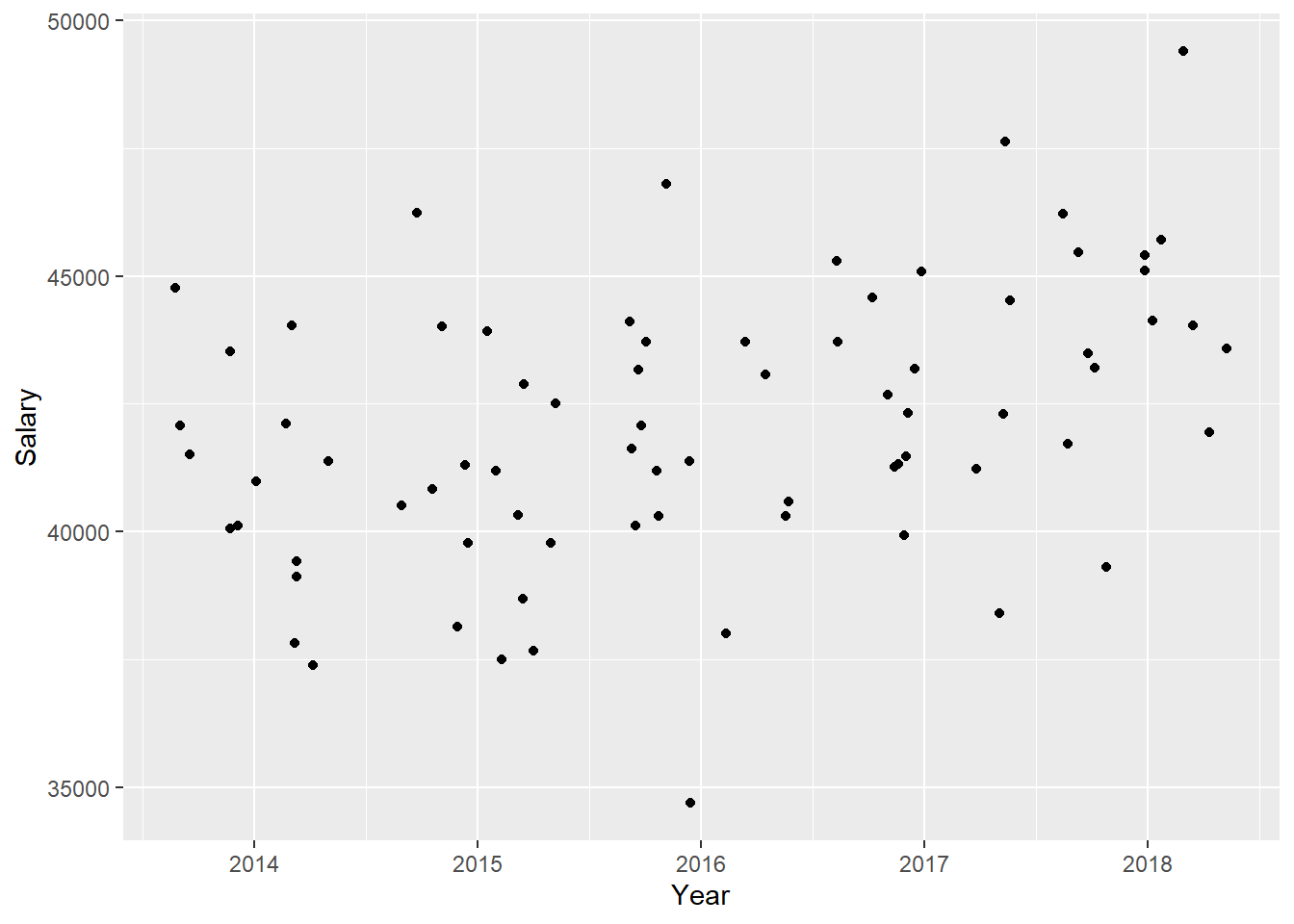Figure 24: The significance of the year on the salary for engineers, Year 2014 - 2018

p1 <- plot_model (model, type = "pred", terms = c("sex"))

p2 <- tb_unique %>%
ggplot () +
geom_jitter (mapping = aes(x = sex, y = salary)) +
labs(
x = "Sex",
y = "Salary"
)

gridExtra::grid.arrange(p1, p2, ncol = 2)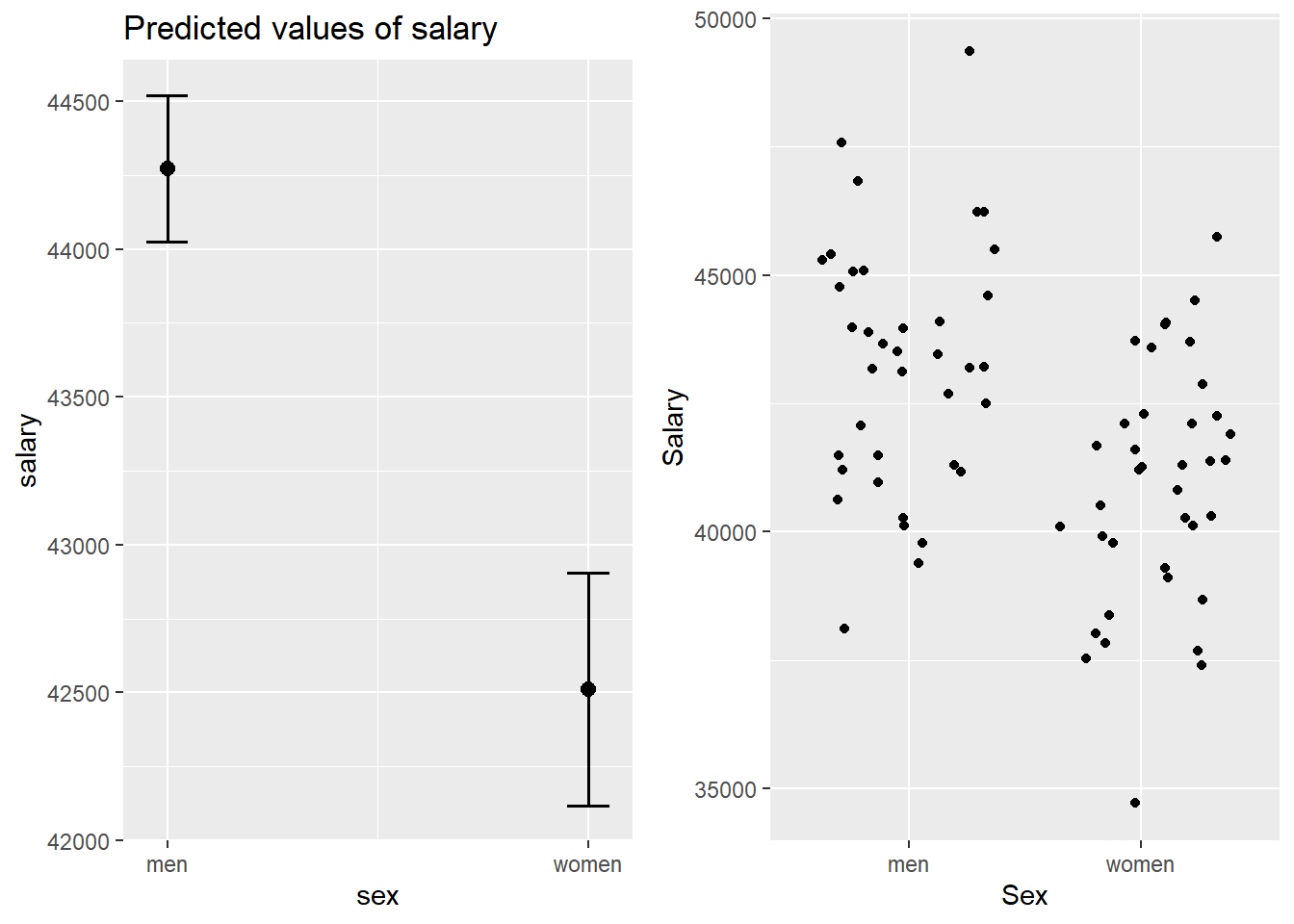Figure 25: The significance of gender on the salary for engineers, Year 2014 - 2018

p1 <- plot_model (model, type = "pred", terms = c("suming"))

p2 <- partial(tbnum_bag, pred.var = "suming") %>% autoplot()

gridExtra::grid.arrange(p1, p2, ncol = 2)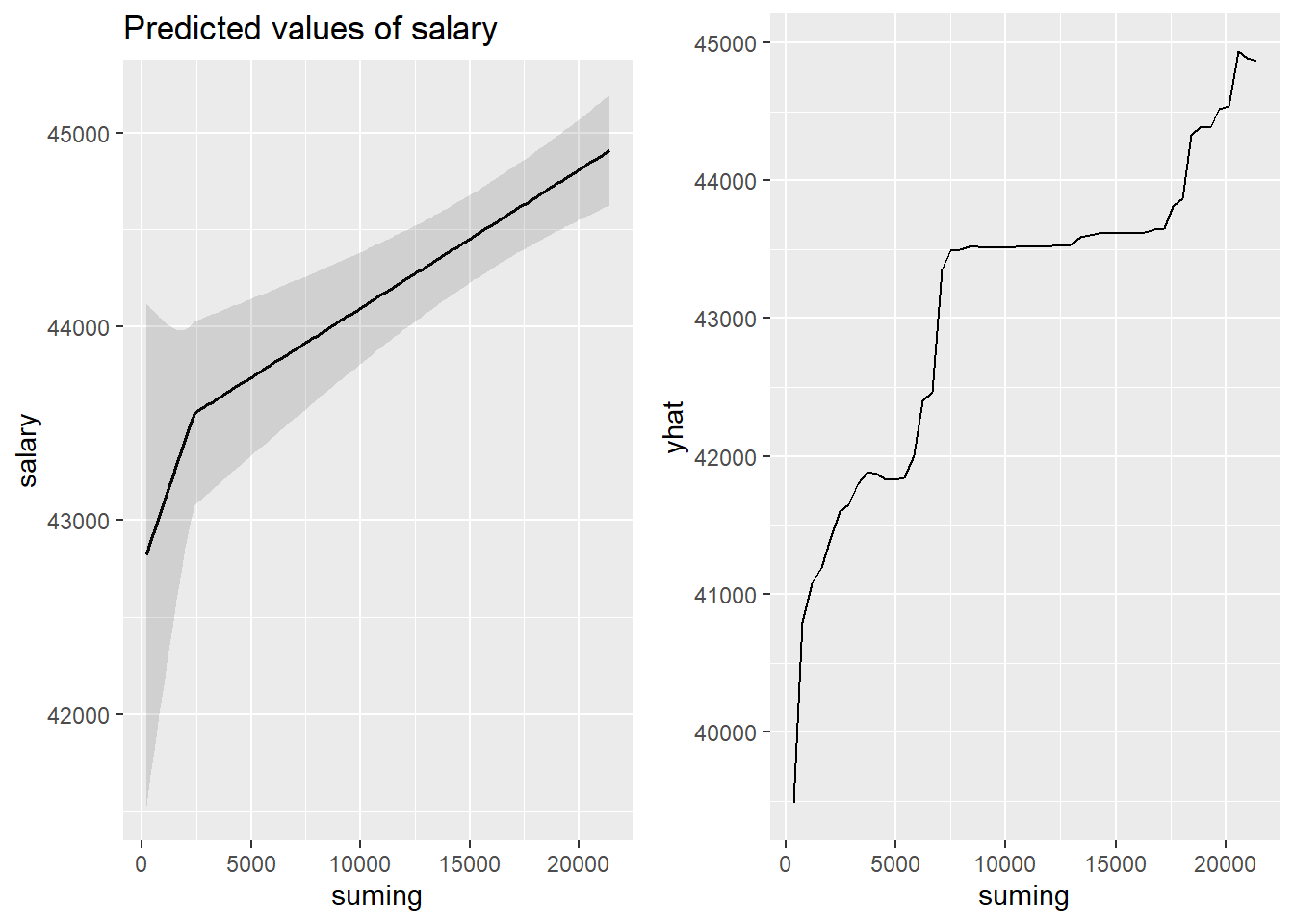Figure 26: The significance of the number of engineers on the salary for engineers, Year 2014 - 2018

tb_unique %>%
ggplot () +
geom_jitter (mapping = aes(x = suming, y = salary)) +
labs(
x = "# engineers in the region",
y = "Salary"
)Figure 27: The significance of the number of engineers on the salary for engineers, Year 2014 - 2018

p1 <- plot_model (model, type = "pred", terms = c("salaryquotient", "sex"))

p2 <- tb_unique %>%
ggplot () +
geom_jitter (mapping = aes(x = salaryquotient, y = salary, colour = sex)) +
labs(
x = "Quotient between salary for men and women",
y = "Salary"
)

gridExtra::grid.arrange(p1, p2, ncol = 2)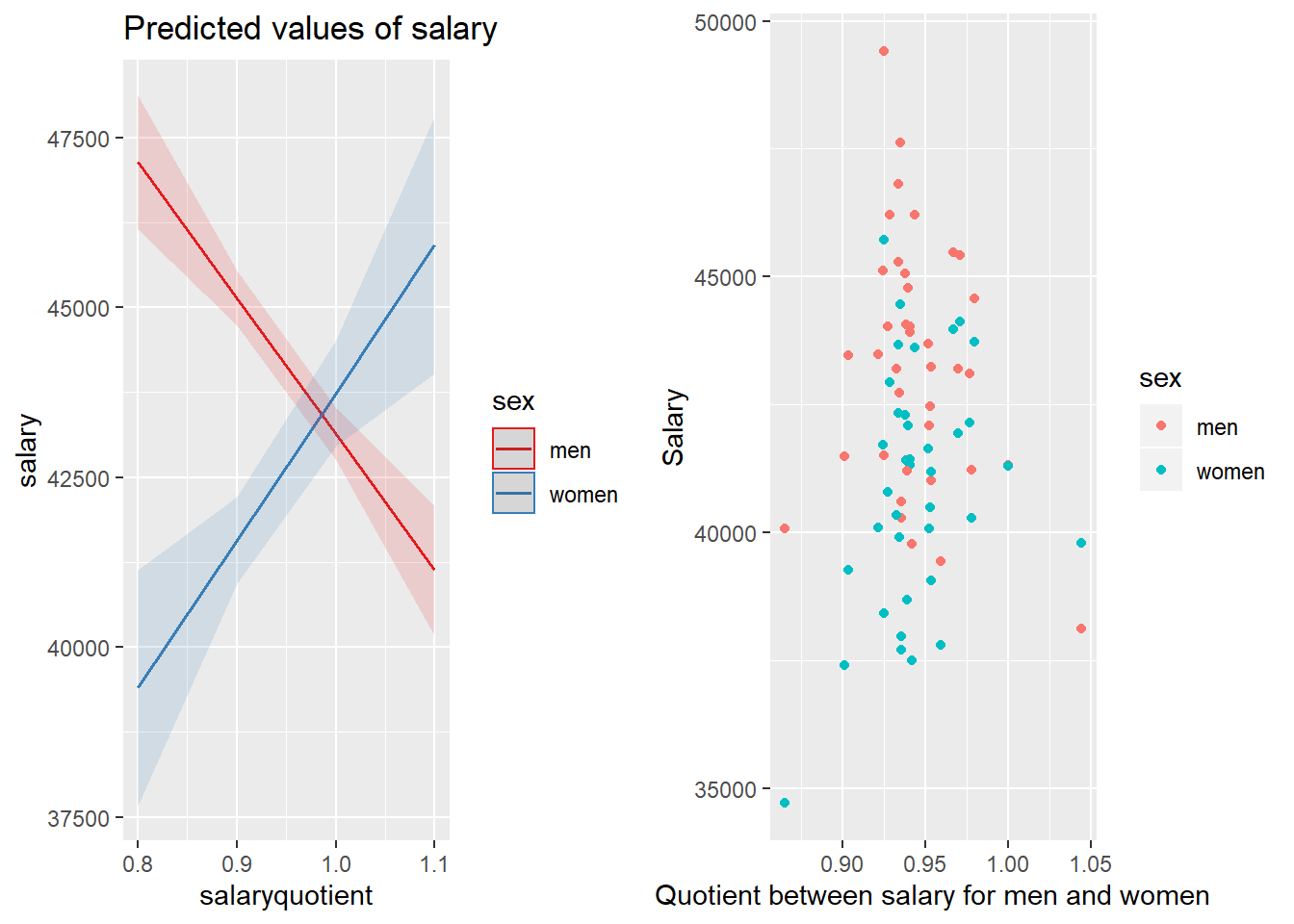Figure 28: The significance of the interaction between sex and the quotient between salary for men and women within each group defined by year and region on the salary for engineers, Year 2014 - 2018

p1 <- plot_model (model, type = "pred", terms = c("year_n", "sex"))

p2 <- tb_unique %>%
ggplot () +
geom_jitter (mapping = aes(x = year_n, y = salary, colour = sex)) +
labs(
x = "Year",
y = "Salary"
)

gridExtra::grid.arrange(p1, p2, ncol = 2)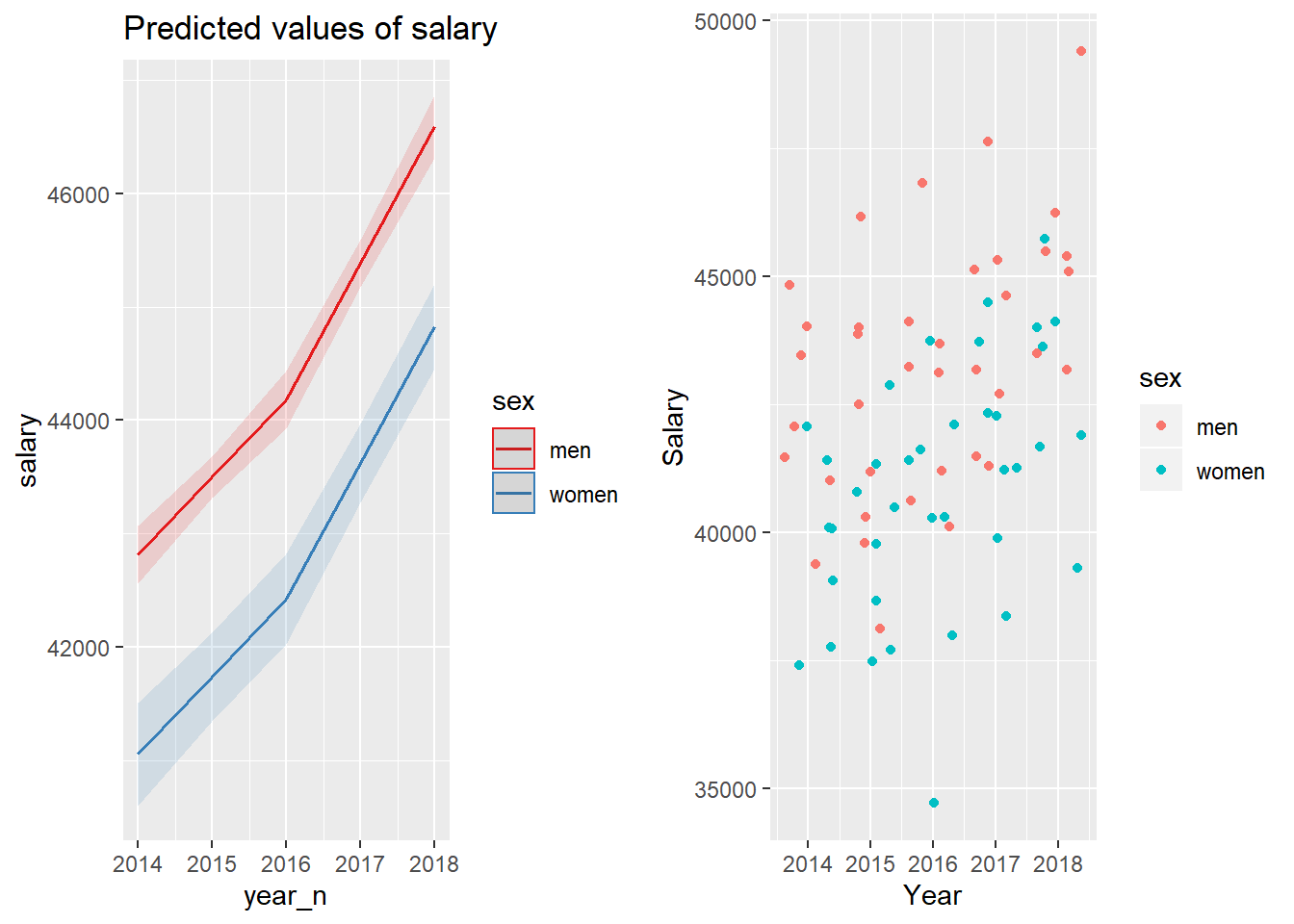Figure 29: The combination of the year and sex on the salary for engineers, Year 2014 - 2018

p1 <- plot_model (model, type = "pred", terms = c("perc_women_region", "sex"))

p2 <- tb_unique %>%
ggplot () +
geom_jitter (mapping = aes(x = perc_women_region, y = salary, colour = sex)) +
labs(
x = "Percent women in region",
y = "Salary"
)

gridExtra::grid.arrange(p1, p2, ncol = 2)Figure 30: The combination of the per cent women in the region and sex on the salary for engineers, Year 2014 - 2018

p1 <- plot_model (model, type = "pred", terms = c("suming", "sex"))

p2 <- tb_unique %>%
ggplot () +
geom_jitter (mapping = aes(x = suming, y = salary, colour = sex)) +
labs(
x = "# engineers in the region",
y = "Salary"
)

gridExtra::grid.arrange(p1, p2, ncol = 2)Figure 31: The combination of the number of engineers in the region and sex on the salary for engineers, Year 2014 - 2018

p1 <- plot_model (model, type = "pred", terms = c("year_n", "perc_women_region"))

p2 <- partial(tbnum_bag, pred.var = c("perc_women_region", "year_n")) %>%
plotPartial(levelplot = FALSE, zlab = "yhat", drape = TRUE,
colorkey = TRUE, screen = list(z = -20, x = -60))

gridExtra::grid.arrange(p1, p2, ncol = 2)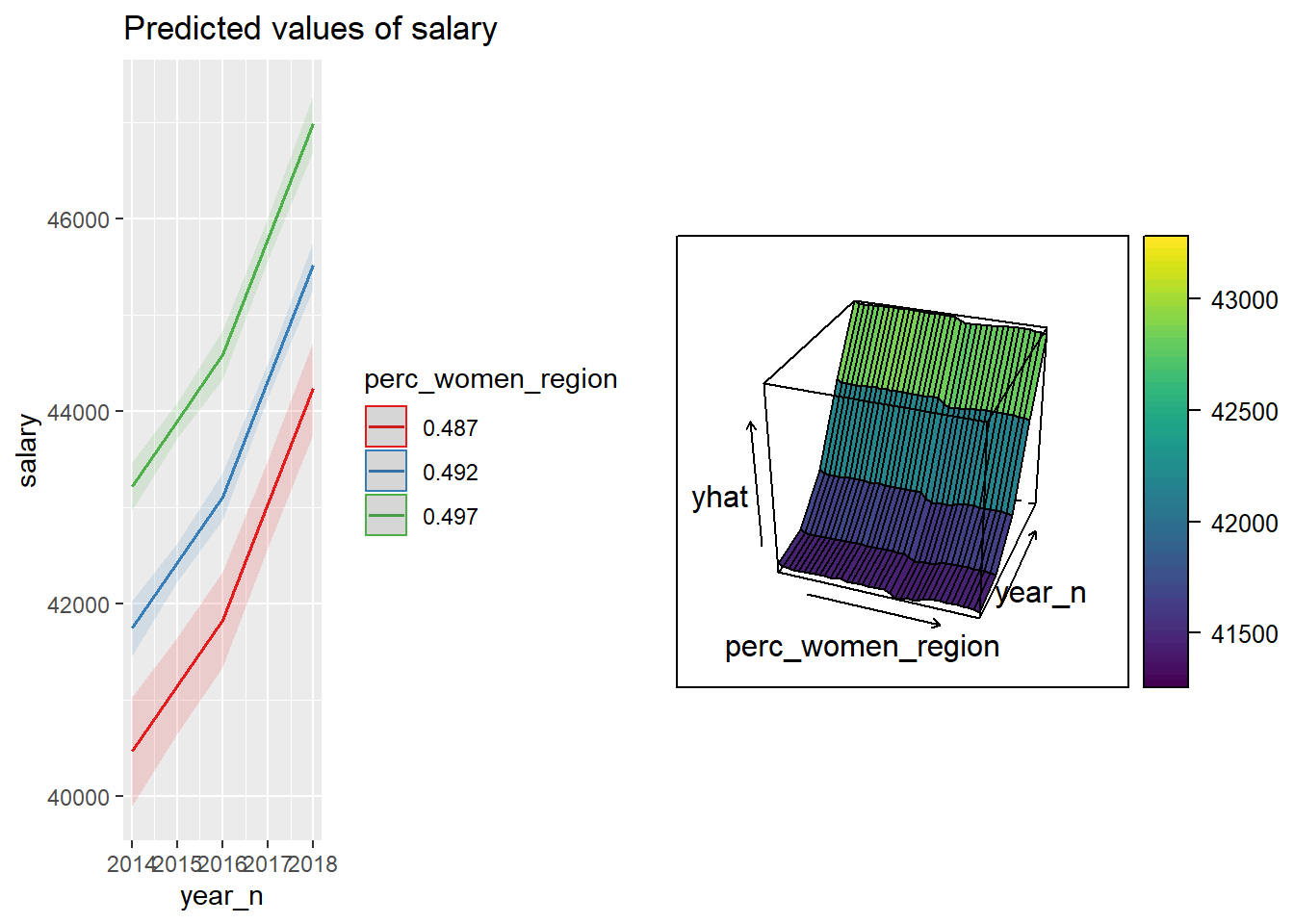Figure 32: The combination of the year and per cent women in the region on the salary for engineers, Year 2014 - 2018

tb_unique %>%
ggplot () +
geom_jitter (mapping = aes(x = year_n, y = salary, colour = perc_women_region)) +
labs(
x = "Year",
y = "Salary"
)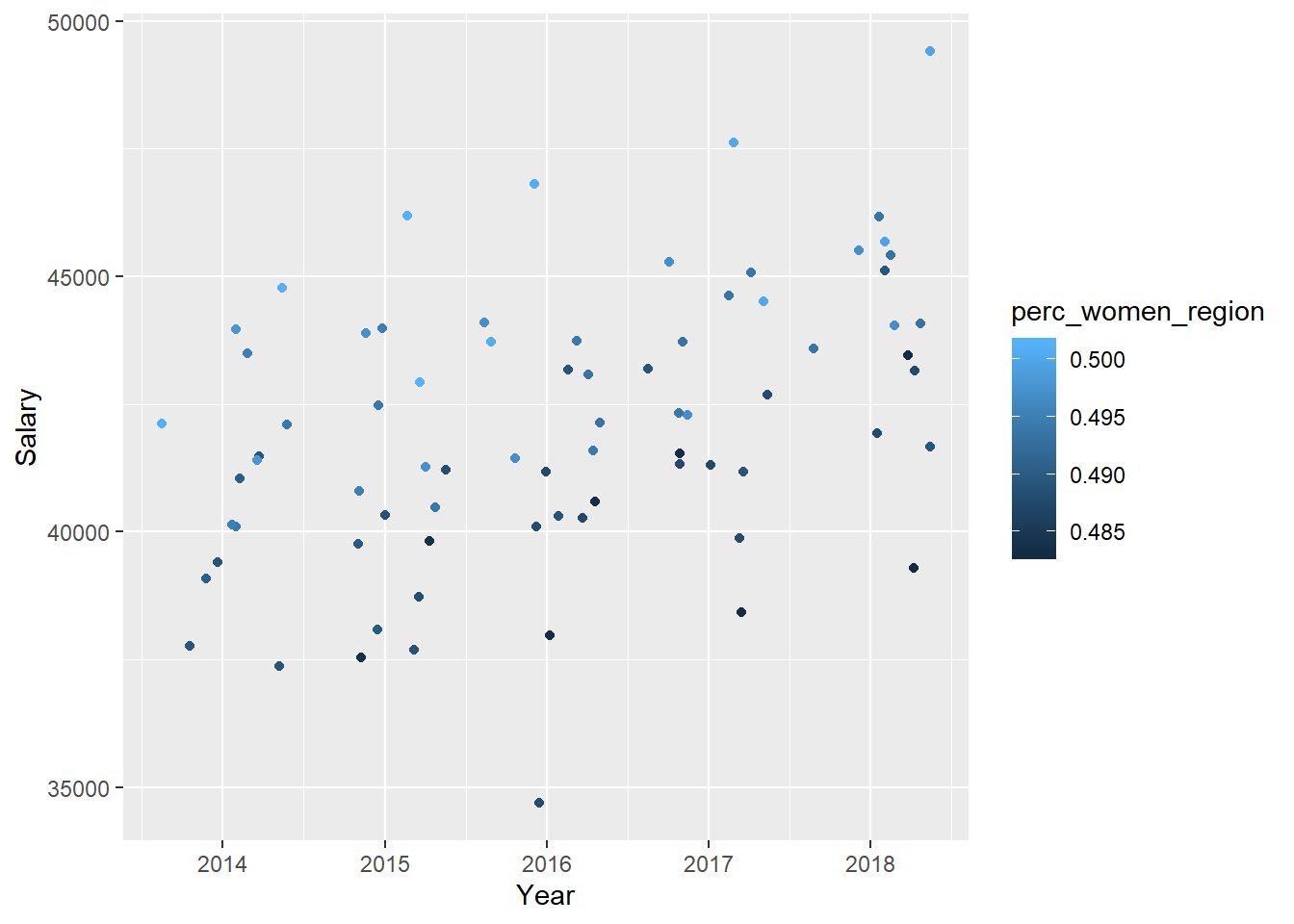Figure 33: The combination of the year and per cent women in the region on the salary for engineers, Year 2014 - 2018

p1 <- plot_model (model, type = "pred", terms = c("year_n", "suming"))

p2 <- partial(tbnum_bag, pred.var = c("suming", "year_n")) %>%
plotPartial(levelplot = FALSE, zlab = "yhat", drape = TRUE,
colorkey = TRUE, screen = list(z = -20, x = -60))

gridExtra::grid.arrange(p1, p2, ncol = 2)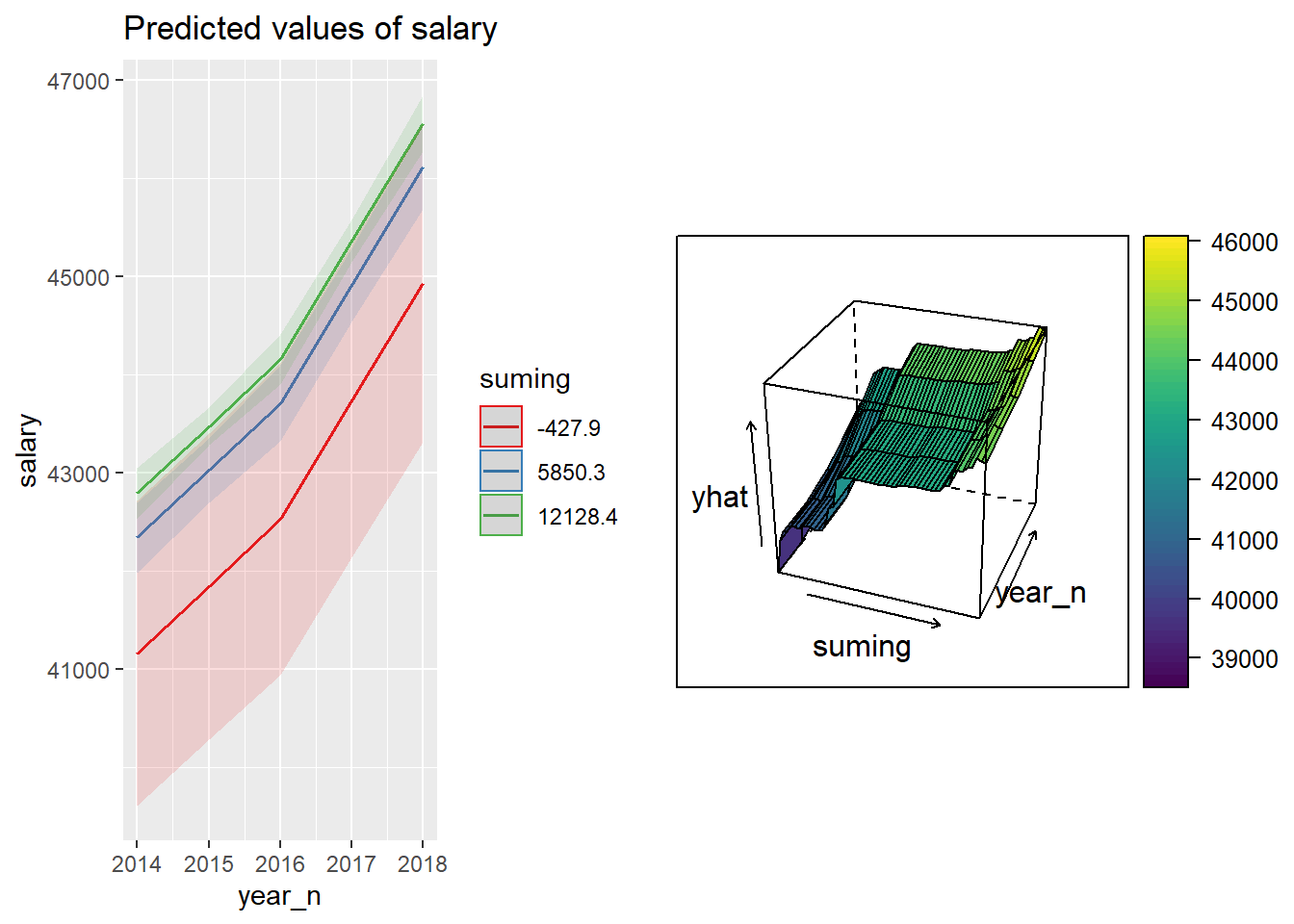Figure 34: The combination of the year and number of engineers in the region on the salary for engineers, Year 2014 - 2018

tb_unique %>%
ggplot () +
geom_jitter (mapping = aes(x = year_n, y = salary, colour = suming)) +
labs(
x = "Year",
y = "Salary"
)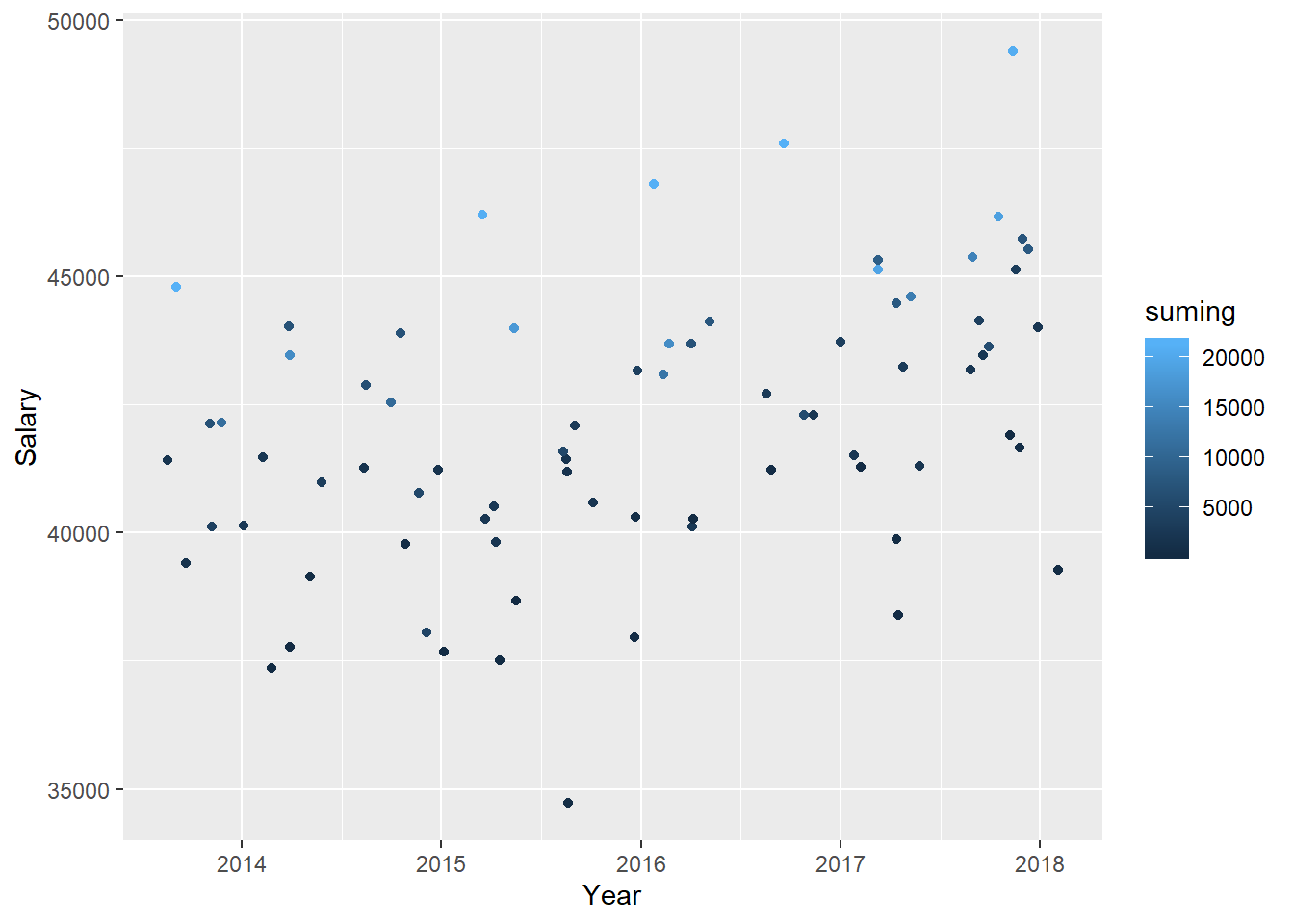Figure 35: The combination of the year and number of engineers in the region on the salary for engineers, Year 2014 - 2018

p1 <- plot_model (model, type = "pred", terms = c("suming", "perc_women_region"))

p2 <- partial(tbnum_bag, pred.var = c("perc_women_region", "suming")) %>%
plotPartial(levelplot = FALSE, zlab = "yhat", drape = TRUE,
colorkey = TRUE, screen = list(z = -20, x = -60))

gridExtra::grid.arrange(p1, p2, ncol = 2)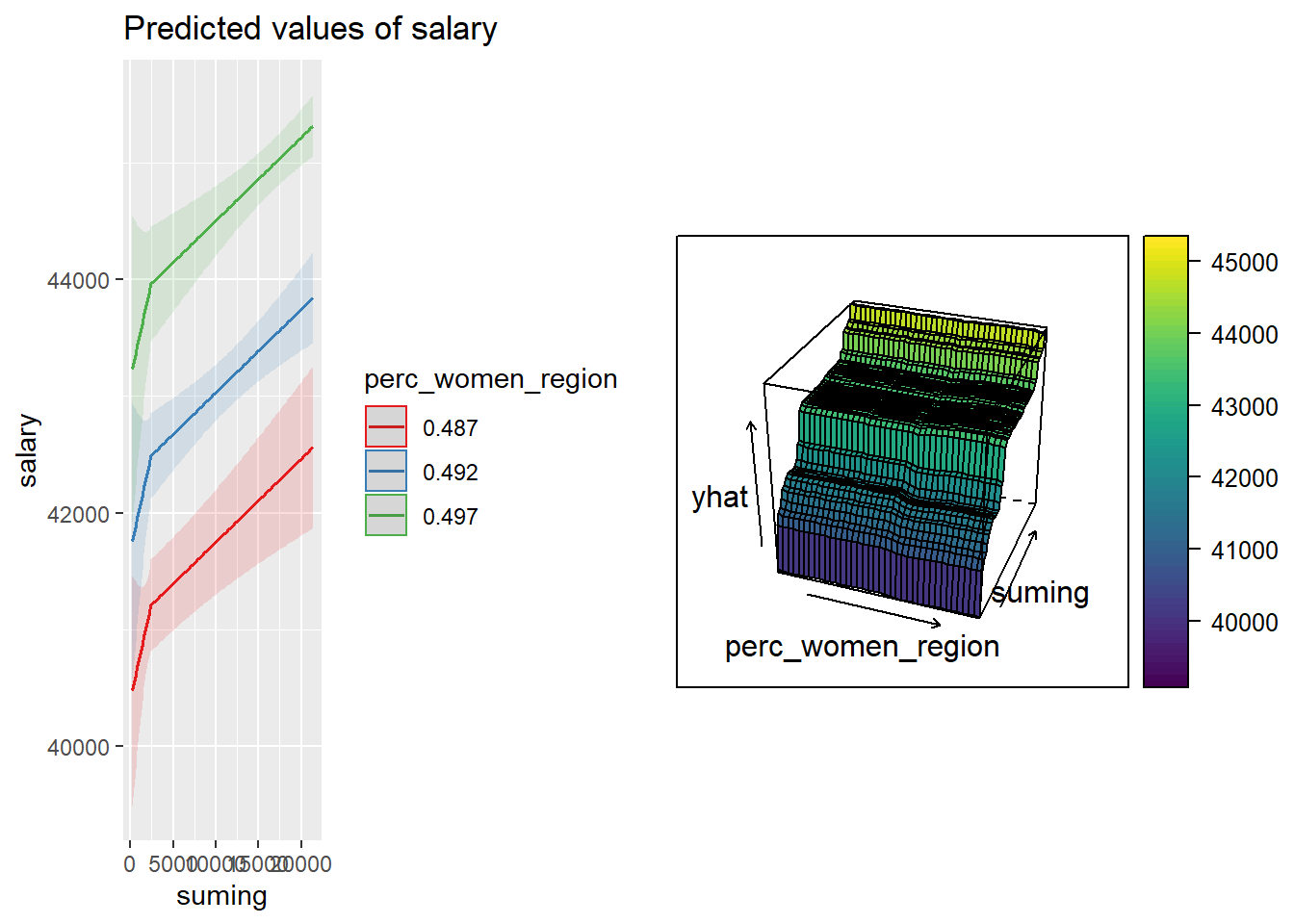Figure 36: The combination of the number of engineers in the region and per cent women in the region on the salary for engineers, Year 2014 - 2018

tb_unique %>%
ggplot () +
geom_jitter (mapping = aes(x = suming, y = salary, colour = perc_women_region)) +
labs(
x = "# engineers in the region",
y = "Salary"
)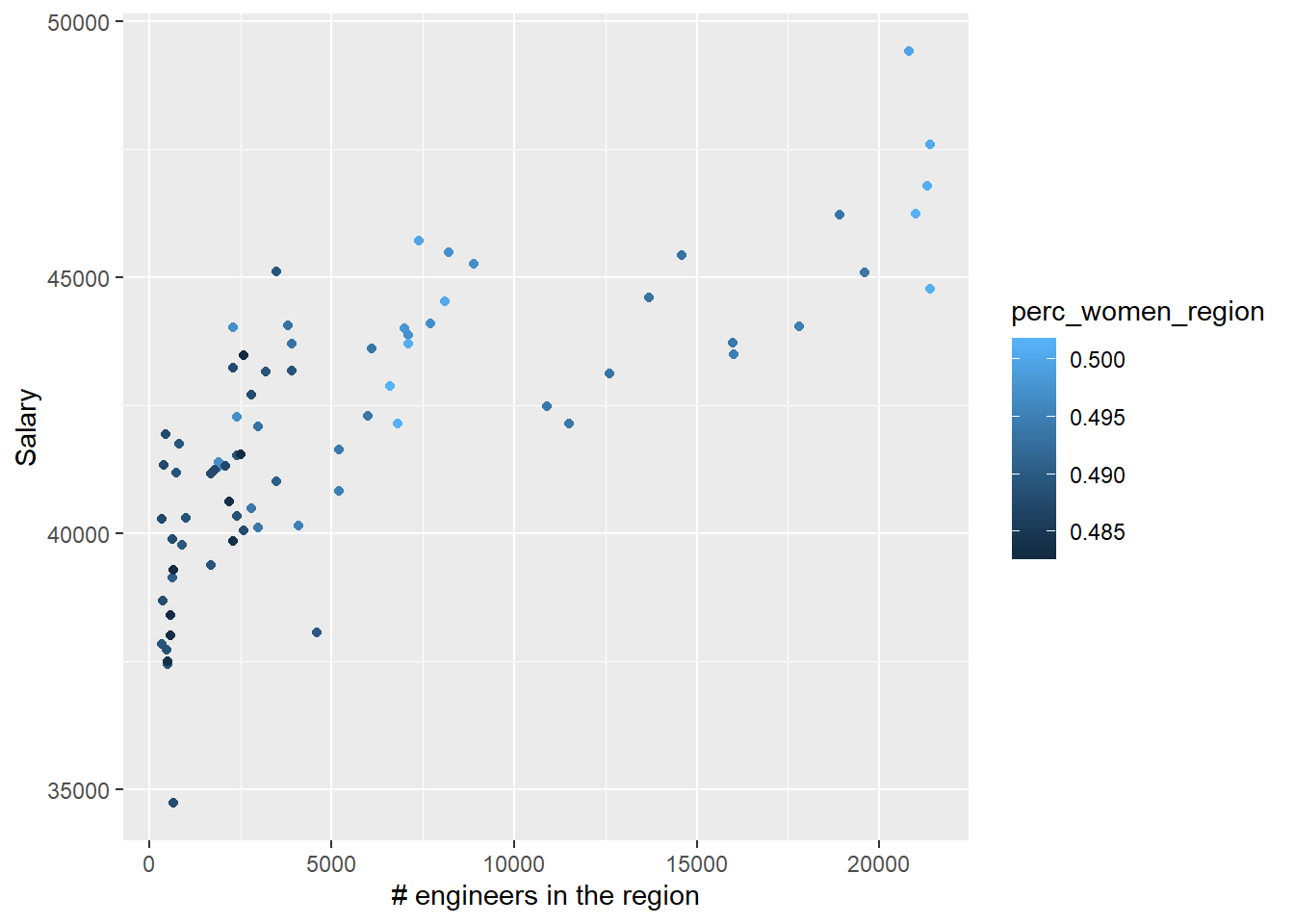Figure 37: The combination of the number of engineers in the region and per cent women in the region on the salary for engineers, Year 2014 - 2018